# hana_ml.visualizers package¶

The Visualizers Package consists of the following sections:

## hana_ml.visualizers.eda¶

This module represents an eda plotter. Matplotlib is used for all visualizations.

hana_ml.visualizers.eda.quarter_plot(data, col, key=None, ax=None)

Perform quarter plot to view the seasonality.

Parameters

Input data.

colstr

Name of the time series data column.

keystr, optional

Name of the ID column.

Defaults to the index column of `data` (i.e. data.index) if it is set.

axAxes, optional

The axes for the plot.

Returns
axAxes

The axes for the plot.

Examples

```>>> quarter_plot(data=df, col="Y", key="ID")
```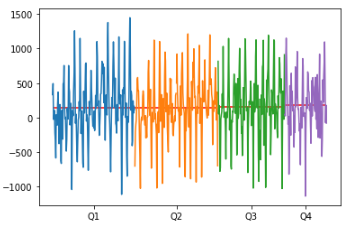hana_ml.visualizers.eda.seasonal_plot(data, col, key=None, ax=None)

Plot time series data by year.

Parameters

Input data.

colstr

Name of the time series data column.

keystr, optional

Name of the ID column.

Defaults to the index column of `data` (i.e. data.index) if it is set.

axAxes, optional

The axes for the plot.

Returns
axAxes

The axes for the plot.

Examples

```>>> seasonal_plot(data=df, col="Y", key="ID")
```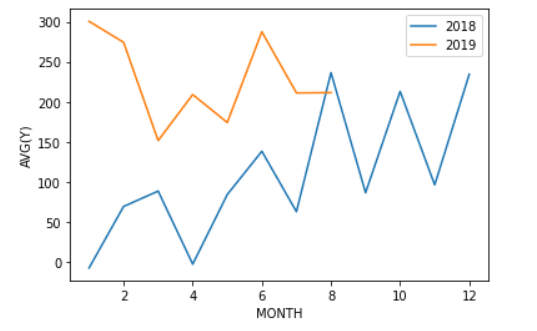hana_ml.visualizers.eda.timeseries_box_plot(data, col, key=None, ax=None, cycle='MONTH')

Plot year-wise/month-wise box plot.

Parameters

Input data.

colstr

Name of the time series data column.

keystr, optional

Name of the ID column.

Defaults to the index column of `data` (i.e. data.index) if it is set.

axAxes, optional

The axes for the plot.

cycle{"YEAR", "QUARTER", "MONTH", "WEEK"}, optional

It defines the x-axis for the box plot.

Defaults to "MONTH".

Returns
axAxes

The axes for the plot.

Examples

Example 1: YEAR

```>>> timeseries_box_plot(data=df, col="Y", key="ID", cycle="YEAR")
```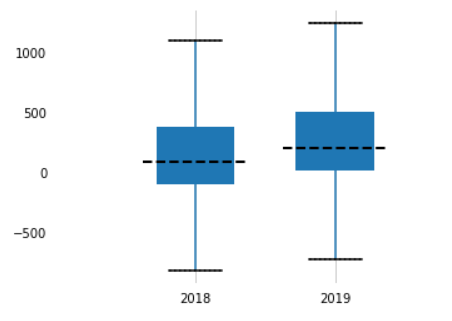Example 2: MONTH

```>>> timeseries_box_plot(data=df, col="Y", key="ID", cycle="MONTH")
```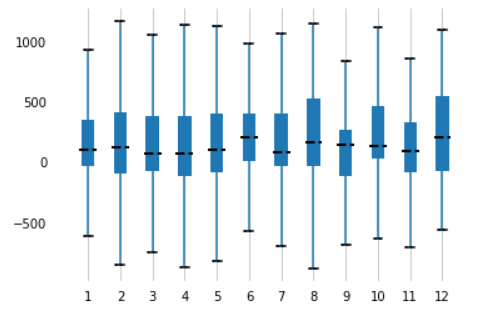Example 3: QUARTER

```>>> timeseries_box_plot(data=df, col="Y", key="ID", cycle="QUARTER")
```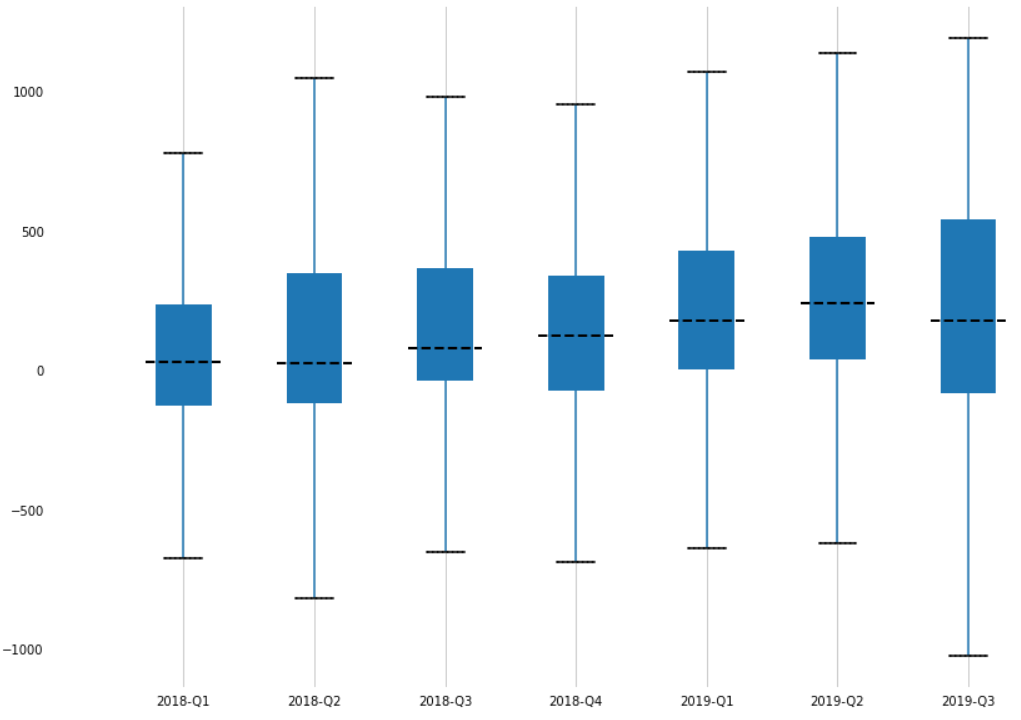hana_ml.visualizers.eda.plot_acf(data, col, key=None, thread_ratio=None, method=None, max_lag=None, ax=None, calculate_confint=True, alpha=None, bartlett=None, title=None)

Plot autocorrelation function (PACF).

Parameters

Input data.

colstr

Name of the time series data column.

keystr, optional

Name of the ID column.

Defaults to the index column of `data` (i.e. data.index) if it is set.

The ratio of available threads.

• 0: single thread

• 0~1: percentage

• Others: heuristically determined

Valid only when `method` is set as 'brute_force'.

Defaults to -1.

method{'auto', 'brute_force', 'fft'}, optional

Indicates the method to be used to calculate the correlation function.

Defaults to 'auto'.

max_lagint, optional

Maximum lag for the correlation function.

Defaults to sqrt(n), where n is the data number.

axAxes

The axes for the plot.

calculate_confintbool, optional

Controls whether to calculate confidence intervals or not.

If it is True, two additional columns of confidence intervals are shown in the result.

Defaults to True.

alphafloat, optional

Confidence bound for the given level are returned. For instance if alpha=0.05, 95 % confidence bound is returned.

Valid only when only `calculate_confint` is True.

Defaults to 0.05.

bartlettbool, optional
• False: using standard error to calculate the confidence bound.

• True: using Bartlett's formula to calculate confidence bound.

Valid only when only `calculate_confint` is True.

Defaults to True.

titlestr, optional

The title of plot.

Defaults to "Autocorrelation".

Returns
axAxes

The axes for the plot.

Examples

```>>> plot_acf(df, key='ID', col='ts', method = 'fft', thread_ratio=0.4, calculate_confint=True, max_lag=40)
```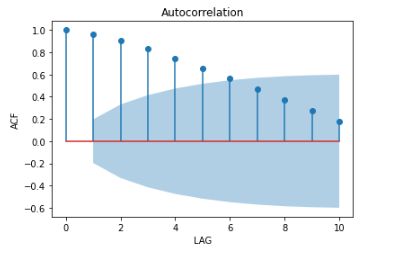hana_ml.visualizers.eda.plot_pacf(data, col, key=None, thread_ratio=None, method=None, max_lag=None, ax=None, calculate_confint=True, alpha=None, bartlett=None, title=None)

Plot partial autocorrelation function (PACF).

Parameters

Input data.

colstr, optional

Name of the time series data column.

keystr, optional

Name of the ID column.

Defaults to the index column of `data` (i.e. data.index) if it is set.

The ratio of available threads.

• 0: single thread

• 0~1: percentage

• Others: heuristically determined

Valid only when `method` is set as 'brute_force'.

Defaults to -1.

method{'auto', 'brute_force', 'fft'}, optional

Indicates the method to be used to calculate the correlation function.

Defaults to 'auto'.

max_lagint, optional

Maximum lag for the correlation function.

Defaults to sqrt(n), where n is the data number.

axAxes

The axes for the plot.

calculate_confintbool, optional

Controls whether to calculate confidence intervals or not.

If it is True, two additional columns of confidence intervals are shown in the result.

Defaults to True.

alphafloat, optional

Confidence bound for the given level are returned. For instance if alpha=0.05, 95 % confidence bound is returned.

Valid only when only `calculate_confint` is True.

Defaults to 0.05.

bartlettbool, optional
• False: using standard error to calculate the confidence bound.

• True: using Bartlett's formula to calculate confidence bound.

Valid only when only `calculate_confint` is True.

Defaults to True.

titlestr, optional

The title of plot.

Defaults to "Partial Autocorrelation".

Returns
axAxes

The axes for the plot.

Examples

```>>> plot_pacf(df, key='ID', col='ts', method = 'fft', thread_ratio=0.4, max_lag=20, calculate_confint=True)
```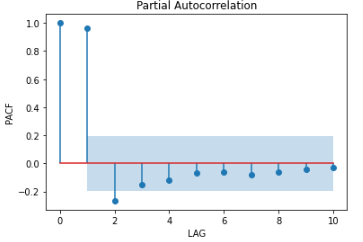hana_ml.visualizers.eda.plot_time_series_outlier(data, col, key=None, window_size=None, detect_seasonality=None, alpha=None, periods=None, outlier_method=None, threshold=None, title=None, ax=None)

Perform OutlierDetectionTS and plot time series with the highlighted outliers.

Parameters

Input data containing the target time-series.

`data` should have at least two columns: one is ID column, the other is raw data.

colstr, optional

Name of the time series data column.

keystr, optional

Name of the ID column.

Defaults to the index column of `data` (i.e. data.index) if it is set.

window_sizeint, optional

Odd number, the window size for median filter, not less than 3.

Defaults to 3.

outlier_methodstr, optional

The method for calculate the outlier score from residual. - 'z1' : Z1 score. - 'z2' : Z2 score. - 'iqr' : IQR score. - 'mad' : MAD score.

Defaults to 'z1'.

thresholdfloat, optional

The threshold for outlier score. If the absolute value of outlier score is beyond the threshold, we consider the corresponding data point as an outlier.

Defaults to 3.

detect_seasonalitybool, optional

When calculating the residual,

• False: Does not consider the seasonal decomposition.

• True: Considers the seasonal decomposition.

Defaults to False.

alphafloat, optional

The criterion for the autocorrelation coefficient. The value range is (0, 1). A larger value indicates a stricter requirement for seasonality.

Only valid when `detect_seasonality` is True.

Defaults to 0.2.

periodsint, optional

When this parameter is not specified, the algorithm will search the seasonal period. When this parameter is specified between 2 and half of the series length, autocorrelation value is calculated for this number of periods and the result is compared to `alpha` parameter. If correlation value is equal to or higher than `alpha`, decomposition is executed with the value of `periods`. Otherwise, the residual is calculated without decomposition. For other value of parameter `periods`, the residual is also calculated without decomposition.

No Default value.

The ratio of available threads.

• 0: single thread.

• 0~1: percentage.

• Others: heuristically determined.

Only valid when `detect_seasonality` is True.

Defaults to -1.

titlestr, optional

The title of plot.

Defaults to "Outliers".

axAxes, optional

The axes for the plot.

Returns
axesAxes

The axes for the plot.

Examples

```>>> plot_time_series_outlier(data=df, key='ID', col='ts')
```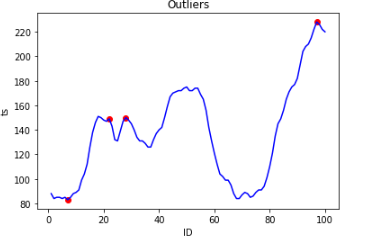hana_ml.visualizers.eda.plot_moving_average(data, col, rolling_window, key=None, ax=None, compare=True)

Plot the rolling mean by given rolling window size.

Parameters

Input data.

colstr, optional

Name of the time series data column.

rolling_windowint, optional

Window size for rolling function. If negative, it will use the points before CURRENT ROW.

keystr, optional

Name of the ID column.

Defaults to the index column of `data` (i.e. data.index) if it is set.

axAxes, optional

The axes for the plot.

comparebool, optional

If True, it will plot the data and its moving average. Otherwise, only moving average will be plotted.

Defaults to True.

Returns
axAxes

The axes for the plot.

Examples

```>>> plot_moving_average(data=df, key='ID', col='ts', rolling_window=10)
```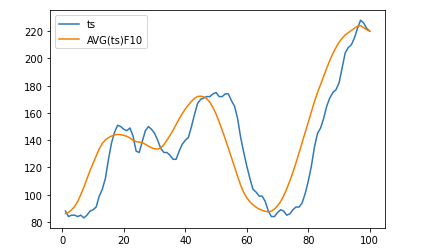hana_ml.visualizers.eda.plot_rolling_stddev(data, col, rolling_window, key=None, ax=None)

Plot the rolling standard deviation by given rolling window size.

Parameters

Input data.

colstr, optional

Name of the time series data column.

rolling_windowint, optional

Window size for rolling function. If negative, it will use the points before CURRENT ROW.

keystr, optional

Name of the ID column.

Defaults to the index column of `data` (i.e. data.index) if it is set.

axAxes

The axes for the plot.

Returns
axAxes

The axes for the plot.

Examples

```>>> plot_rolling_stddev(data=df, key='ID', col='ts', rolling_window=10)
```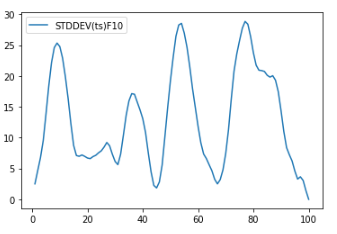hana_ml.visualizers.eda.plot_seasonal_decompose(data, col, key=None, alpha=None, thread_ratio=None, decompose_type=None, extrapolation=None, smooth_width=None, axes=None)

Plot the seasonal decomposition.

Parameters

Input data.

colstr, optional

Name of the time series data column.

keystr, optional

Name of the ID column.

Defaults to the index column of `data` (i.e. data.index) if it is set.

alphafloat, optional

The criterion for the autocorrelation coefficient. The value range is (0, 1). A larger value indicates stricter requirement for seasonality.

Defaults to 0.2.

Controls the proportion of available threads to use. The ratio of available threads.

• 0: single thread.

• 0~1: percentage.

• Others: heuristically determined.

Defaults to -1.

decompose_type{'additive', 'multiplicative', 'auto'}, optional

Specifies decompose type.

• 'multiplicative': Multiplicative decomposition model.

• 'auto': Decomposition model automatically determined from input data.

Defaults to 'auto'.

extrapolationbool, optional

Specifies whether to extrapolate the endpoints. Set to True when there is an end-point issue.

Defaults to False.

smooth_widthint, optional

Specifies the width of the moving average applied to non-seasonal data. 0 indicates linear fitting to extract trends. Can not be larger than half of the data length.

Defaults to 0.

axesAxes array, optional

The axes for the plot.

Returns
axesAxes

The axes for the plot.

Examples

```>>> plot_seasonal_decompose(data=df, col='ts', key= 'ID')
```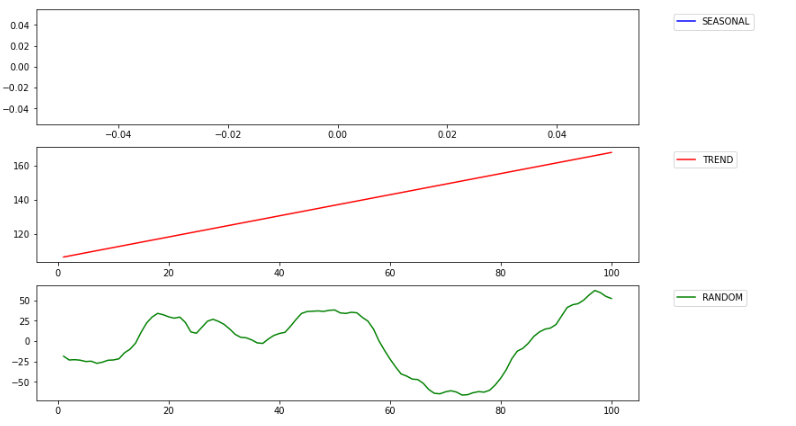hana_ml.visualizers.eda.kdeplot(data, key, features=None, kde=<hana_ml.algorithms.pal.kernel_density.KDE object>, points=1000, enable_plotly=False, **kwargs)

Display a kernel density estimate plot for SAP HANA DataFrame.

Parameters

Dataframe including the data of density distribution.

keystr

Name of the ID column in the data.

featuresstr/list of str, optional

Name of the feature columns in the data.

kdehana_ml.algorithms.pal.kernel_density.KDE, optional

KDE Calculation.

Defaults to KDE().

pointsint, optional

The number of points for plotting.

Defaults to 1000.

enable_plotlybool, optional

Use plotly instead of matplotlib.

Defaults to False.

Returns
axAxes

The axes for the plot.

surfPoly3DCollection

The surface plot object. Only valid for 2D plotting. Only for matplotlib plot.

Examples

```>>> f = plt.figure(figsize=(19, 10))
>>> ax = kdeplot(data, key="PASSENGER_ID", features=["AGE"])
>>> ax.grid()
>>> plt.show()
```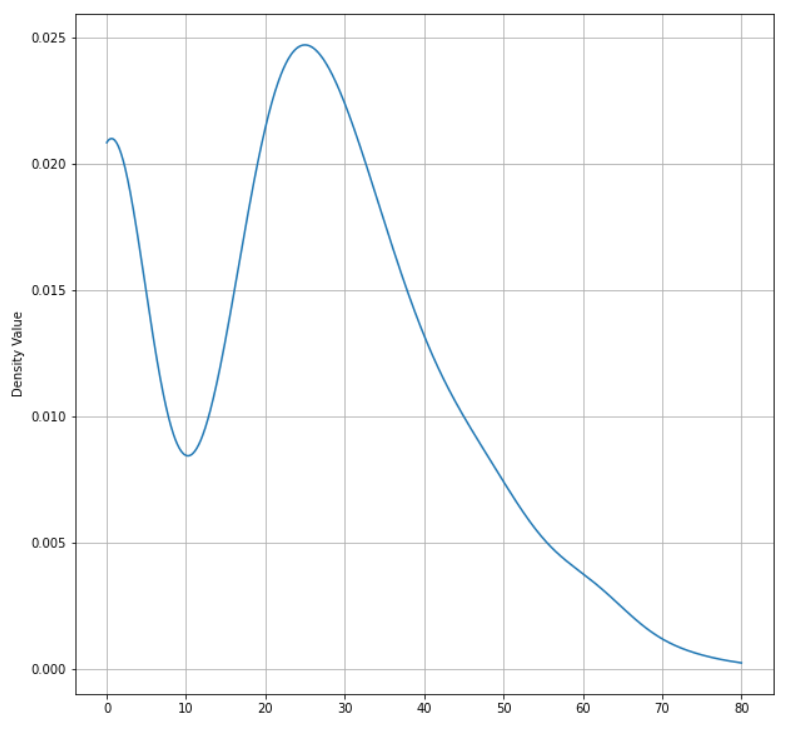```>>> f = plt.figure(figsize=(19, 10))
>>> ax, surf = kdeplot(data, key="PASSENGER_ID", features=["AGE", "FARE"])
>>> ax.grid()
>>> plt.show()
```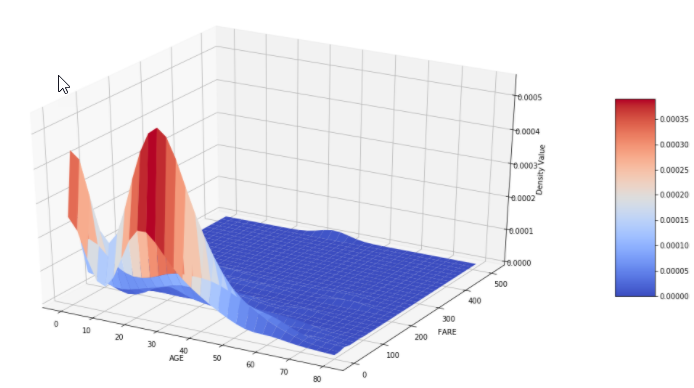hana_ml.visualizers.eda.hist(data, columns, bins=None, debrief=False, x_axis_fontsize=10, x_axis_rotation=0, title_fontproperties=None, default_bins=20, rounding_precision=3, replacena=0, enable_plotly=False, **kwargs)

Plot histograms for SAP HANA DataFrame.

Parameters

DataFrame used for the plot.

columnslist of str

Columns in the DataFrame being plotted.

binsint or dict, optional

The number of bins to create based on the value of column.

Defaults to 20.

debriefbool, optional

Whether to include the skewness debrief.

Defaults to False.

x_axis_fontsizeint, optional

The size of x axis labels.

Defaults to 10.

x_axis_rotationint, optional

The rotation of x axis labels.

Defaults to 0.

title_fontpropertiesFontProperties, optional

Change the font properties for title. Only for Matplotlib plot.

Defaults to None.

default_binsint, optional

The number of bins to create for the column that has not been specified in bins when bins is dict.

Defaults to 20.

debriefbool, optional

Whether to include the skewness debrief.

Defaults to False.

rounding_precisionint, optional

The rounding precision for bin size.

Defaults to 3.

replacenafloat, optional

Replace na with the specified value.

Defaults to 0.

enable_plotlybool, optional

Use plotly instead of matplotlib.

Defaults to False.

Examples

```>>> hist(data=data, columns=['PCLASS', 'AGE', 'SIBSP', 'PARCH', 'FARE'], default_bins=20, bins={"AGE": 10})
```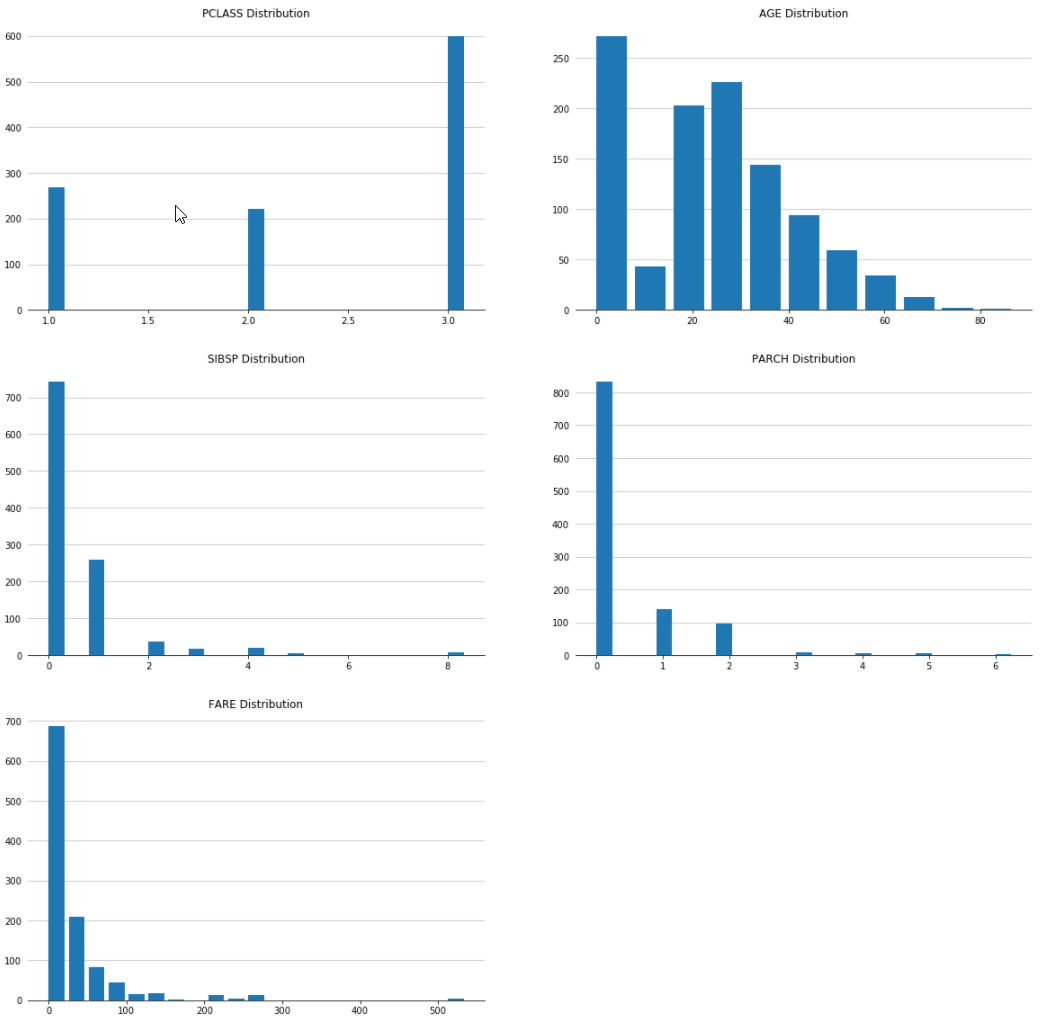class hana_ml.visualizers.eda.EDAVisualizer(ax=None, size=None, cmap=None, enable_plotly=False, fig=None)

Bases: `hana_ml.visualizers.visualizer_base.Visualizer`

Class for all EDA visualizations, including:

• Distribution plot

• Pie plot

• Correlation plot

• Scatter plot

• Bar plot

• Box plot

Parameters
axmatplotlib.Axes, optional

The axes used to plot the figure. Only for matplotlib plot.

Default value is current axes.

sizetuple of integers, optional

(width, height) of the plot in dpi. Only for matplotlib plot.

Default value is the current size of the plot.

cmapmatplotlib.pyplot.colormap, optional

Color map used for the plot. Only for matplotlib plot.

Defaults to None.

enable_plotlybool, optional

Use plotly instead of matplotlib.

Defaults to False.

figFigure, optional

Plotly's figure. Only for plotly plot.

Examples

```>>> f = plt.figure(figsize=(10,10))
>>> ax1 = f.add_subplot(111)
>>> eda = EDAVisualizer(ax1)
```
Attributes
`ax`

Returns the matplotlib Axes where the Visualizer will draw.

`cmap`

Returns the color map being used for the plot.

`size`

Returns the size of the plot in pixels.

Methods

 `bar_plot`(data, column, aggregation[, title, ...]) Displays a bar plot for the SAP HANA DataFrame column specified. `box_plot`(data, column[, outliers, title, ...]) Displays a box plot for the SAP HANA DataFrame column specified. `correlation_plot`(data[, key, corr_cols, ...]) Displays a correlation plot for the SAP HANA DataFrame columns specified. `distribution_plot`(data, column, bins[, ...]) Displays a distribution plot for the SAP HANA DataFrame column specified. `pie_plot`(data, column[, explode, title, ...]) Displays a pie plot for the SAP HANA DataFrame column specified. Reset. `scatter_plot`(data, x, y[, x_bins, y_bins, ...]) Displays a scatter plot for the SAP HANA DataFrame columns specified. `set_ax`(ax) Sets the Axes `set_cmap`(cmap) Sets the colormap `set_size`(size) Sets the size
distribution_plot(data, column, bins, title=None, x_axis_fontsize=10, x_axis_rotation=0, debrief=False, rounding_precision=3, title_fontproperties=None, replacena=0, x_axis_label='', y_axis_label='', subplot_pos=(1, 1), **kwargs)

Displays a distribution plot for the SAP HANA DataFrame column specified.

Parameters

DataFrame used for the plot.

columnstr

Column in the DataFrame being plotted.

binsint

Number of bins to create based on the value of column.

titlestr, optional

Title for the plot.

x_axis_fontsizeint, optional

Size of x axis labels.

Defaults to 10.

x_axis_rotationint, optional

Rotation of x axis labels.

Defaults to 0.

debriefbool, optional

Whether to include the skewness debrief.

Defaults to False.

rounding_precisionint, optional

The rounding precision for bin size.

Defaults to 3.

title_fontpropertiesFontProperties, optional

Change the font properties for title.

Defaults to None.

replacenafloat, optional

Replace na with the specified value.

Defaults to 0.

x_axis_labelstr, optional

x axis label. Only for plotly plot.

Defaults to "".

y_axis_labelstr, optional

y axis label.

Defaults to "". Only for plotly plot.

subplot_postuple, optional

(row, col) for plotly subplot.

Defaults to (1, 1).

Returns
matplotlib:
axAxes

The axes for the plot.

bin_datapandas.DataFrame

The data used in the plot.

plotly:
figFigure

The distribution plot.

trace: graph object trace

The trace of the plot, used in hist().

bin_datapandas.DataFrame

The data used in the plot.

Examples

```>>> f = plt.figure(figsize=(35, 10))
>>> ax1 = f.add_subplot(111)
>>> eda = EDAVisualizer(ax1)
>>> ax1, dist_data = eda.distribution_plot(data=data, column="FARE", bins=100, title="Distribution of FARE")
>>> plt.show()
```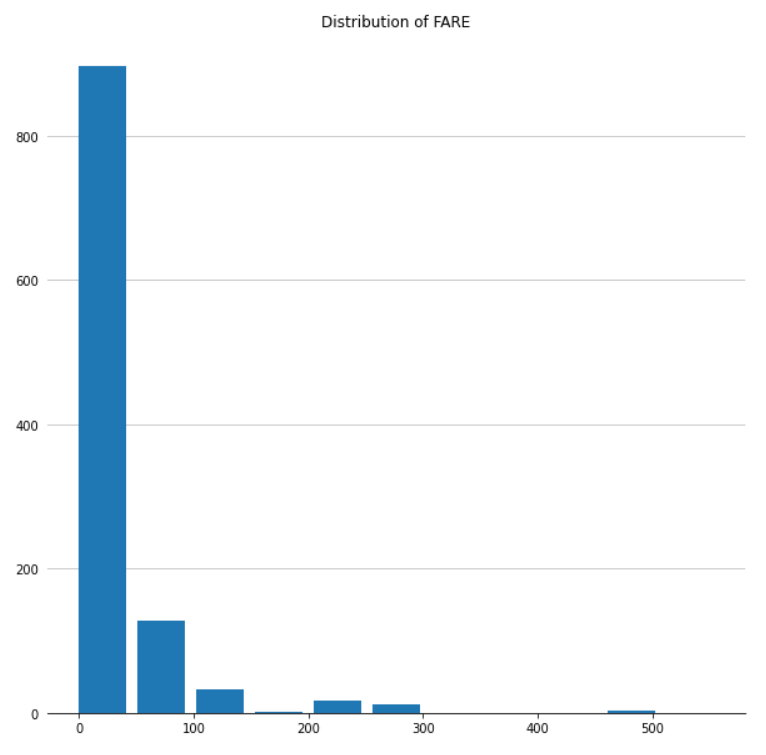pie_plot(data, column, explode=0.03, title=None, legend=True, title_fontproperties=None, legend_fontproperties=None, subplot_pos=(1, 1), **kwargs)

Displays a pie plot for the SAP HANA DataFrame column specified.

Parameters

DataFrame used for the plot.

columnstr

Column in the DataFrame being plotted.

explodefloat, optional

Relative spacing between pie segments. Only for matplotlib plot.

titlestr, optional

Title for the plot.

Defaults to None.

legendbool, optional

Whether to show the legend for the plot. Only for matplotlib plot.

Defaults to True.

title_fontpropertiesFontProperties, optional

Change the font properties for title. Only for matplotlib plot.

Defaults to None.

legend_fontpropertiesFontProperties, optional

Change the font properties for legend. Only for matplotlib plot.

Defaults to None.

subplot_postuple, optional

(row, col) for plotly subplot.

Defaults to (1, 1).

Returns
matplotlib:
axAxes

The axes for the plot. This can be used to set specific properties for the plot.

pie_datapandas.DataFrame

The data used in the plot.

plotly:
figFigure

The pie plot.

pie_datapandas.DataFrame

The data used in the plot.

Examples

```>>> f = plt.figure(figsize=(8, 8))
>>> ax1 = f.add_subplot(111)
>>> eda = EDAVisualizer(ax1)
>>> ax1, pie_data = eda.pie_plot(data, column="PCLASS", title="% of passengers in each cabin")
>>> plt.show()
```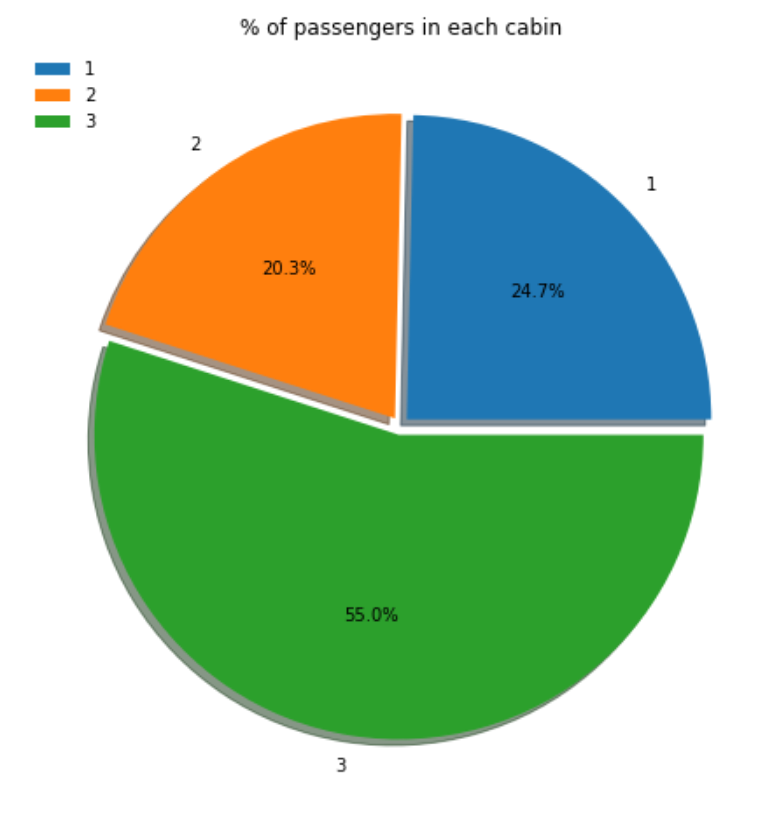correlation_plot(data, key=None, corr_cols=None, label=True, cmap=None, title="Pearson's correlation (r)", **kwargs)

Displays a correlation plot for the SAP HANA DataFrame columns specified.

Parameters

DataFrame used for the plot.

keystr, optional

Name of ID column.

Defaults to None.

corr_colslist of str, optional

Columns in the DataFrame being plotted. If None then all numeric columns will be plotted.

Defaults to None.

labelbool, optional

Plot a colorbar. Only for matplotlib plot.

Defaults to True.

cmapmatplotlib.pyplot.colormap or str, optional

Color map used for the plot.

Defaults to "RdYlBu" for matplotlib and "blues" for plotly.

titlestr, optional

Title of the plot.

Defaults to "Pearson's correlation (r)".

Returns
matplotlib:
axAxes

The axes for the plot. This can be used to set specific properties for the plot.

corrpandas.DataFrame

The data used in the plot.

plotly:
figFigure

The correlation plot.

corrpandas.DataFrame

The data used in the plot.

Examples

```>>> f = plt.figure(figsize=(35, 10))
>>> ax1 = f.add_subplot(111)
>>> eda = EDAVisualizer(ax1)
>>> ax1, corr = eda.correlation_plot(data=data, corr_cols=['PCLASS', 'AGE', 'SIBSP', 'PARCH', 'FARE'], cmap="Blues")
>>> plt.show()
```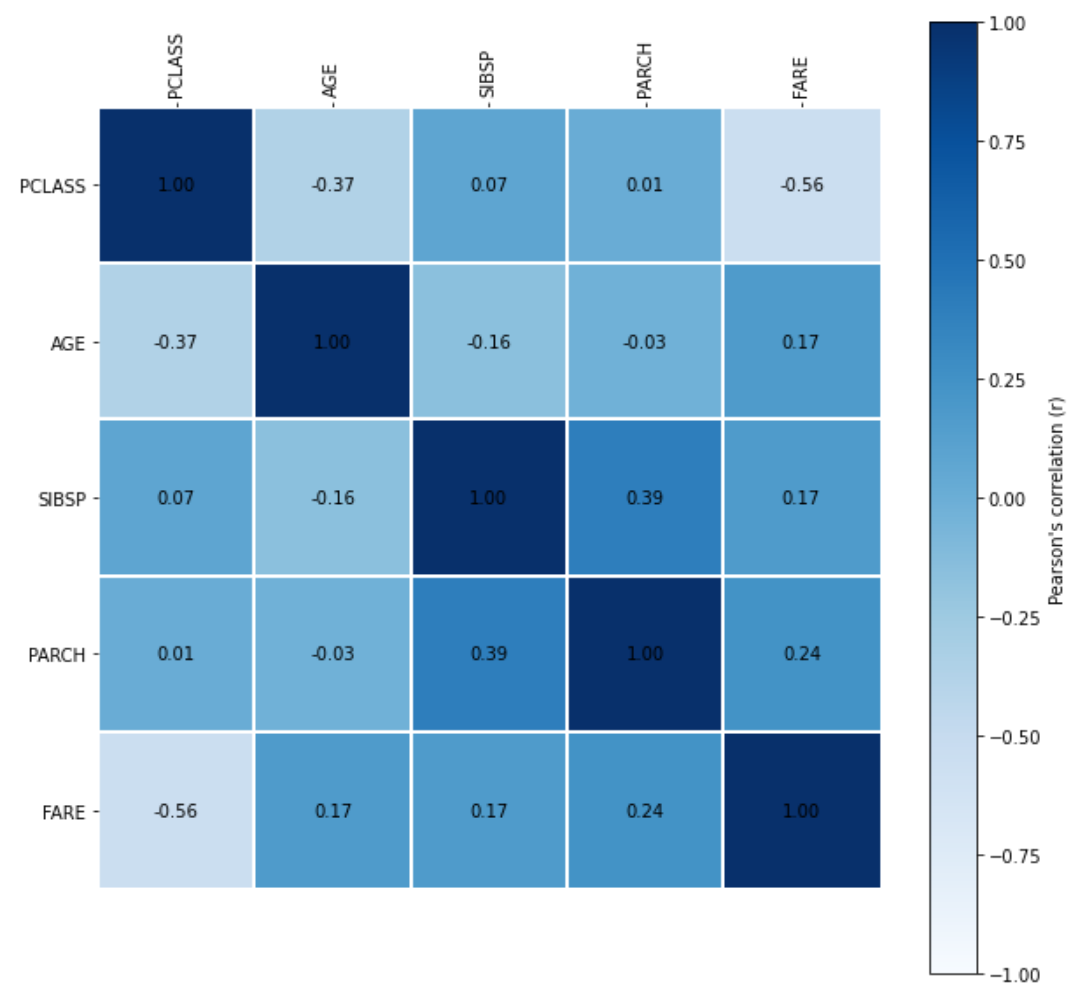scatter_plot(data, x, y, x_bins=None, y_bins=None, title=None, label=None, cmap=None, debrief=True, rounding_precision=3, label_fontsize=12, title_fontproperties=None, sample_frac=1.0, **kwargs)

Displays a scatter plot for the SAP HANA DataFrame columns specified.

Parameters

DataFrame used for the plot.

xstr

Column to be plotted on the x axis.

ystr

Column to be plotted on the y axis.

x_binsint, optional

Number of x axis bins to create based on the value of column.

Defaults to None.

y_binsint

Number of y axis bins to create based on the value of column.

Defaults to None.

titlestr, optional

Title for the plot.

Defaults to None.

labelstr, optional

Label for the color bar.

Defaults to None.

cmapmatplotlib.pyplot.colormap or str, optional

Color map used for the plot.

Defaults to "Blues" for matplotlib and "blues" for plotly.

debriefbool, optional

Whether to include the correlation debrief.

Defaults to True

rounding_precisionint, optional

The rounding precision for bin size. Only for matplotlib plot.

Defaults to 3.

label_fontsizeint, optional

Change the font size for label. Only for matplotlib plot.

Defaults to 12.

title_fontpropertiesFontProperties, optional

Change the font properties for title.

Defaults to None.

sample_fracfloat, optional

Sampling method is applied to data. Valid if x_bins and y_bins are not set.

Defaults to 1.0.

Returns
matplotlib:
axAxes

The axes for the plot.

bin_matrixpandas.DataFrame

The data used in the plot.

plotly:
figFigure

The scatter plot.

Examples

```>>> f = plt.figure(figsize=(10, 10))
>>> ax1 = f.add_subplot(111)
>>> eda = EDAVisualizer(ax1)
>>> ax1, corr = eda.scatter_plot(data=data, x="AGE", y="SIBSP", x_bins=5, y_bins=5)
>>> plt.show()
```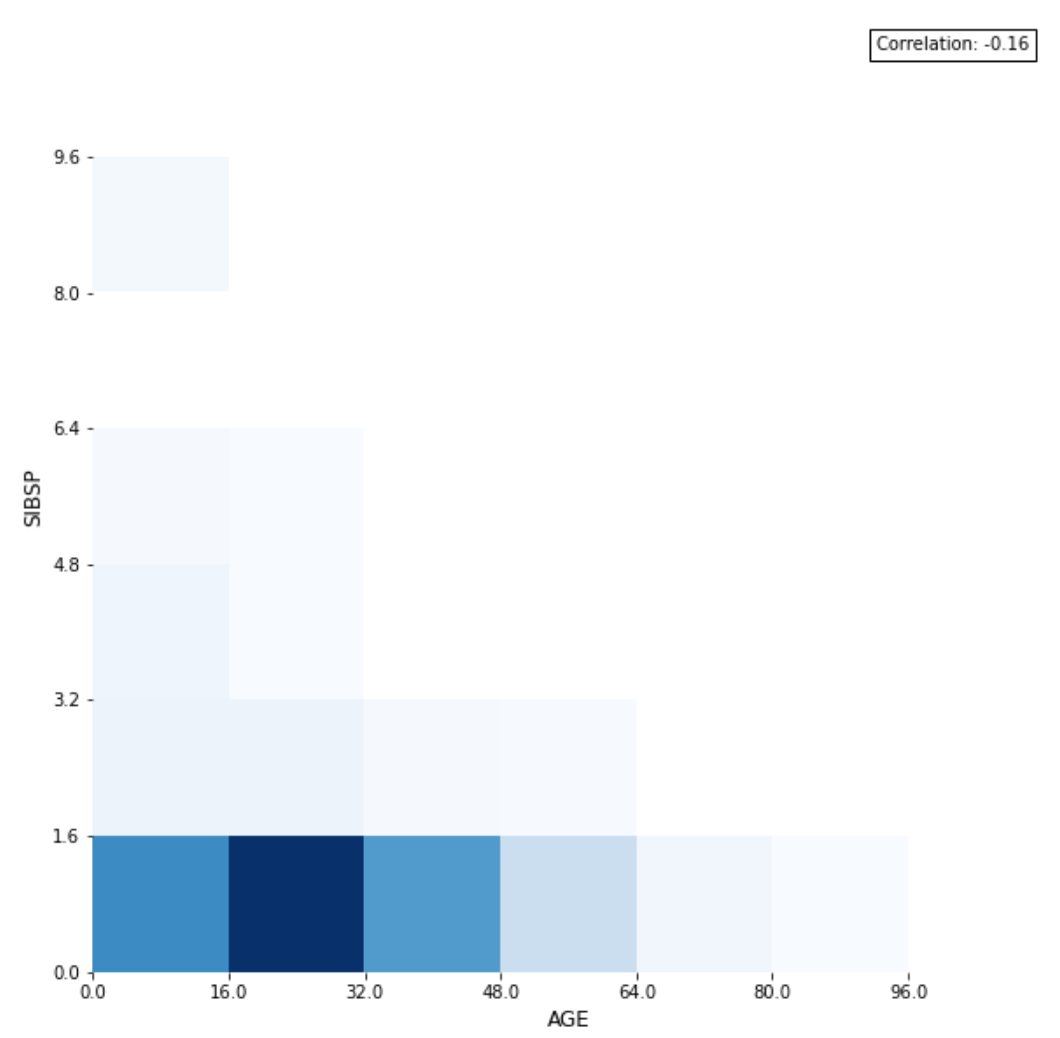```>>> f = plt.figure(figsize=(10, 10))
>>> ax2 = f.add_subplot(111)
>>> eda = EDAVisualizer(ax2)
>>> ax2 = eda.scatter_plot(data=data, x="AGE", y="SIBSP", sample_frac=0.8, s=10, marker='o')
>>> plt.show()
```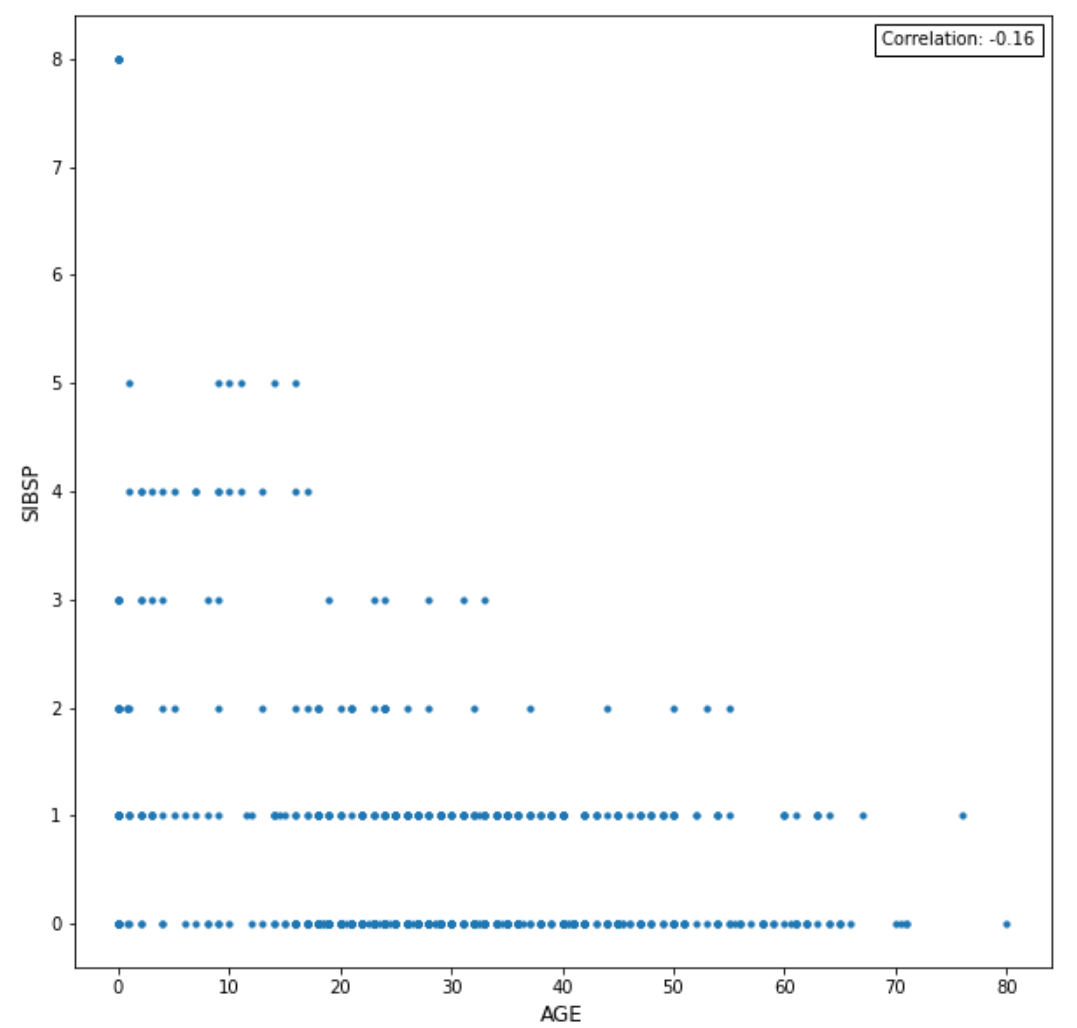bar_plot(data, column, aggregation, title=None, label_fontsize=12, title_fontproperties=None, orientation=None, **kwargs)

Displays a bar plot for the SAP HANA DataFrame column specified.

Parameters

DataFrame used for the plot.

columnstr

Column to be aggregated.

aggregationdict

Aggregation conditions ('avg', 'count', 'max', 'min').

titlestr, optional

Title for the plot.

Defaults to None.

label_fontsizeint, optional

The size of label. Only for matplotlib plot.

Defaults to 12.

title_fontpropertiesFontProperties, optional

Change the font properties for title.

Defaults to None.

orientationstr, optional

One of 'h' for horizontal or 'v' for vertical.

Only valid when plotly plot is enabled.

Defaults to 'v'.

Returns
axAxes

The axes for the plot.

bar_datapandas.DataFrame

The data used in the plot.

Examples

```>>> ax1 = f.add_subplot(111)
>>> eda = EDAVisualizer(ax1)
>>> ax, bar_data = eda.bar_plot(data=data, column='COLUMN', aggregation={'COLUMN':'count'})
```

Returns : bar plot (count) of 'COLUMN'

```>>> ax1 = f.add_subplot(111)
>>> eda = EDAVisualizer(ax1)
>>> ax, bar_data = eda.bar_plot(data=data, column='COLUMN', aggregation={'OTHER_COLUMN':'avg'})
```

Returns : bar plot (avg) of 'COLUMN' against 'OTHER_COLUMN'

box_plot(data, column, outliers=False, title=None, groupby=None, lower_outlier_fence_factor=0, upper_outlier_fence_factor=0, title_fontproperties=None, vert=False, legend=True, multiplier=1.5, **kwargs)

Displays a box plot for the SAP HANA DataFrame column specified.

Parameters

DataFrame used for the plot.

columnstr

Column in the DataFrame being plotted.

outliersbool

Whether to plot suspected outliers and outliers.

Defaults to False.

titlestr, optional

Title for the plot.

Defaults to None.

groupbystr, optional

Column to group by and compare.

Defaults to None.

lower_outlier_fence_factorfloat, optional

The lower bound of outlier fence factor.

Defaults to 0.

upper_outlier_fence_factor

The upper bound of outlier fence factor.

Defaults to 0.

title_fontpropertiesFontProperties, optional

Change the font properties for title.

Defaults to None.

vertbool, optional

Vertical box plot if True.

Defaults to False.

legendbool, optional

Display legend if True. Only available for matplotlib.

Defaults to True.

multiplierfloat, optional

The multiplier used in the IQR test.

Defaults to 1.5.

Returns
axAxes

The axes for the plot.

sta_tablepandas.DataFrame or list of pandas.DataFrame

The data used in the plot.

Examples

```>>> f = plt.figure(figsize=(10, 10))
>>> ax1 = f.add_subplot(111)
>>> eda = EDAVisualizer(ax1)
>>> ax1, corr = eda.box_plot(data=data, column="AGE")
>>> plt.show()
```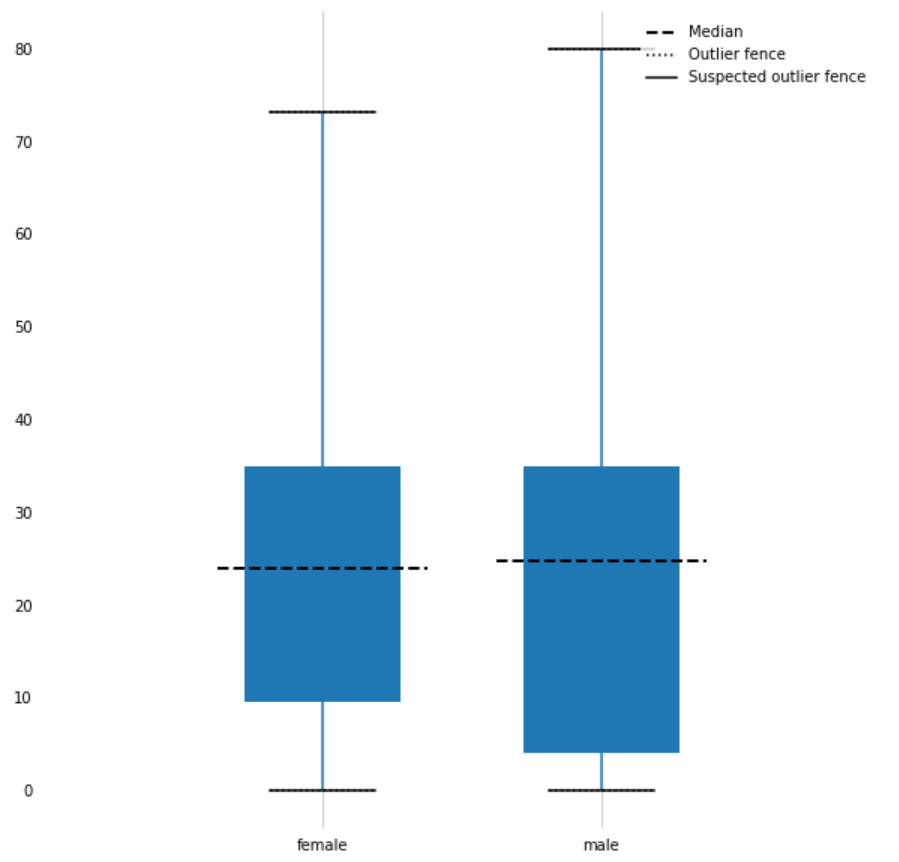```>>> f = plt.figure(figsize=(10, 10))
>>> ax1 = f.add_subplot(111)
>>> eda = EDAVisualizer(ax1)
>>> ax1, corr = eda.box_plot(data=data, column="AGE", groupby="SEX")
>>> plt.show()
```property ax

Returns the matplotlib Axes where the Visualizer will draw.

property cmap

Returns the color map being used for the plot.

reset()

Reset.

set_ax(ax)

Sets the Axes

set_cmap(cmap)

Sets the colormap

set_size(size)

Sets the size

property size

Returns the size of the plot in pixels.

class hana_ml.visualizers.eda.Profiler(*args, **kwargs)

Bases: `object`

A class to build a SAP HANA Profiler, including:

• Variable descriptions

• Missing values %

• High cardinality %

• Skewness

• Numeric distributions

• Categorical distributions

• Correlations

• High correlation warnings

Methods

 `description`(data, key[, bins, ...]) Returns a SAP HANA profiler, including: `set_size`(fig, figsize) Set the size of the data description plot, in inches.
description(data, key, bins=20, missing_threshold=10, card_threshold=100, skew_threshold=0.5, figsize=None)

Returns a SAP HANA profiler, including:

• Variable descriptions

• Missing values %

• High cardinality %

• Skewness

• Numeric distributions

• Categorical distributions

• Correlations

• High correlation warnings

Parameters

DataFrame used for the plat.

keystr, optional

Name of the key column in the DataFrame.

binsint, optional

Number of bins for numeric distributions. Default value = 20.

missing_thresholdfloat

Percentage threshold to display missing values.

card_thresholdint

Threshold for column to be considered with high cardinality.

skew_thresholdfloat

Absolute value threshold for column to be considered as highly skewed.

tight_layoutbool, optional

Use matplotlib tight layout or not.

figsizetuple, optional

Size of figure to be plotted. First element is width, second is height.

Note: categorical columns with cardinality warnings are not plotted.
Returns
figFigure

matplotlib axis of the profiler

set_size(fig, figsize)

Set the size of the data description plot, in inches.

Parameters
figax

The returned axes constructed by the description method.

figsizetuple

Tuple of width and height for the plot.

## hana_ml.visualizers.metrics¶

This module represents a visualizer for metrics.

The following class is available:

class hana_ml.visualizers.metrics.MetricsVisualizer(ax=None, size=None, cmap=None, title=None, enable_plotly=False)

Bases: `hana_ml.visualizers.visualizer_base.Visualizer`, `object`

The MetricVisualizer is used to visualize metrics.

Parameters
axmatplotlib.Axes, optional

The axes to use to plot the figure. Default value : Current axes

sizetuple of integers, optional

(width, height) of the plot in dpi Default value: Current size of the plot.

titlestr, optional

Title for the plot.

enable_plotlybool, optional

Use plotly instead of matplotlib.

Defaults to False.

Attributes
`ax`

Returns the matplotlib Axes where the Visualizer will draw.

`cmap`

Returns the color map being used for the plot.

`size`

Returns the size of the plot in pixels.

Methods

 `plot_confusion_matrix`(df[, normalize]) This function plots the confusion matrix and returns the Axes where this is drawn. Reset. `set_ax`(ax) Sets the Axes `set_cmap`(cmap) Sets the colormap `set_size`(size) Sets the size
plot_confusion_matrix(df, normalize=False, **kwargs)

This function plots the confusion matrix and returns the Axes where this is drawn.

Parameters
dfDataFrame

Data points to the resulting confusion matrix. This dataframe's columns should match columns ('CLASS', '')

property ax

Returns the matplotlib Axes where the Visualizer will draw.

property cmap

Returns the color map being used for the plot.

reset()

Reset.

set_ax(ax)

Sets the Axes

set_cmap(cmap)

Sets the colormap

set_size(size)

Sets the size

property size

Returns the size of the plot in pixels.

## hana_ml.visualizers.m4_sampling¶

This module contains M4 algorithm for sampling query.

The following function is available:

hana_ml.visualizers.m4_sampling.get_min_index(data)

Get Minimum Timestamp of Time Series Data Only for internal use, do not show it in the doc.

Parameters

Time series data whose the 1st column is index and the 2nd one is value.

Returns
datetime

Return the minimum timestamp.

hana_ml.visualizers.m4_sampling.get_max_index(data)

Get Maximum Timestamp of Time Series Data Only for internal use, do not show it in the doc.

Parameters

Time series data whose 1st column is index and 2nd one is value.

Returns
datetime

Return the maximum timestamp.

hana_ml.visualizers.m4_sampling.m4_sampling(data, width)

M4 algorithm for big data visualization

Parameters

Data to be sampled. Time series data whose 1st column is index and 2nd one is value.

widthint

Sampling Rate. It is an indicator of how many pixels being in the picture.

Returns
DataFrame

Return the sampled dataframe.

## hana_ml.visualizers.model_debriefing¶

This module represents a visualizer for tree model.

The following class is available:

class hana_ml.visualizers.model_debriefing.TreeModelDebriefing

Bases: `object`

Visualize tree model.

Examples

Visualize Tree Model in JSON format:

```>>> TreeModelDebriefing.tree_debrief(rdt.model_)
```Visualize Tree Model in DOT format:

```>>> TreeModelDebriefing.tree_parse(rdt.model_)
>>> TreeModelDebriefing.tree_debrief_with_dot(rdt.model_)
```Visualize Tree Model in XML format the model is stored in the dataframe rdt.model_:

```>>> treeModelDebriefing.tree_debrief(rdt.model_)
```Methods

 `shapley_explainer`(reason_code_data, feature_data) Create Shapley explainer to explain the output of machine learning model. `tree_debrief`(model) Visualize tree model by data in JSON or XML format. `tree_debrief_with_dot`(model[, ...]) Visualize tree model by data in DOT format. `tree_export`(model, filename) Save the tree model as a html file. `tree_export_with_dot`(model, filename) Save the tree model as a html file. `tree_parse`(model) Transform tree model content using DOT language.
static tree_debrief(model)

Visualize tree model by data in JSON or XML format.

Parameters
modelDataFrame

Tree model.

Returns
HTML Page

This HTML page can be rendered by browser.

static tree_export(model, filename)

Save the tree model as a html file.

Parameters
modelDataFrame

Tree model.

filenamestr

Html file name.

static tree_parse(model)

Transform tree model content using DOT language.

Parameters
modelDataFrame

Tree model.

static tree_debrief_with_dot(model, iframe_height: int = 800, digraph_config: = None, display=True)

Visualize tree model by data in DOT format.

Parameters
modelDataFrame

Tree model.

iframe_heightint, optional

Frame height.

Defaults to 800.

digraph_configDigraphConfig, optional

Configuration instance of digraph.

Returns
HTML Page

This HTML page can be rendered by browser.

static tree_export_with_dot(model, filename)

Save the tree model as a html file.

Parameters
modelDataFrame

Tree model.

filenamestr

Html file name.

static shapley_explainer(reason_code_data: hana_ml.dataframe.DataFrame, feature_data: hana_ml.dataframe.DataFrame, reason_code_column_name=None, **kwargs)

Create Shapley explainer to explain the output of machine learning model.

It connects optimal credit allocation with local explanations using the classic Shapley values from game theory and their related extensions.

To get an overview of which features are most important for a model we can plot the Shapley values of every feature for every sample.

Parameters

The Dataframe containing only reason code values.

The Dataframe containing only feature values.

Returns
`ShapleyExplainer`

Shapley explainer.

## hana_ml.visualizers.dataset_report¶

class hana_ml.visualizers.dataset_report.DatasetReportBuilder

Bases: `object`

The DatasetReportBuilder instance can analyze the dataset and generate a report in HTML format.

The instance will call the dropna method of DataFrame internally to handle the missing value of dataset.

The generated report can be embedded in a notebook, including:

• Overview
• Dataset Info

• Variable Types

• High Cardinality %

• Highly Skewed Variables

• Sample
• Top ten rows of dataset

• Variables
• Numeric distributions

• Categorical distributions

• Variable statistics

• Data Correlations

• Data Scatter Matrix

Examples

Create a DatasetReportBuilder instance:

```>>> from hana_ml.visualizers.dataset_report import DatasetReportBuilder
>>> datasetReportBuilder = DatasetReportBuilder()
```

Assume the dataset DataFrame is df and then analyze the dataset:

```>>> datasetReportBuilder.build(df, key="ID")
```

Display the dataset report as a notebook iframe.

```>>> datasetReportBuilder.generate_notebook_iframe_report()
```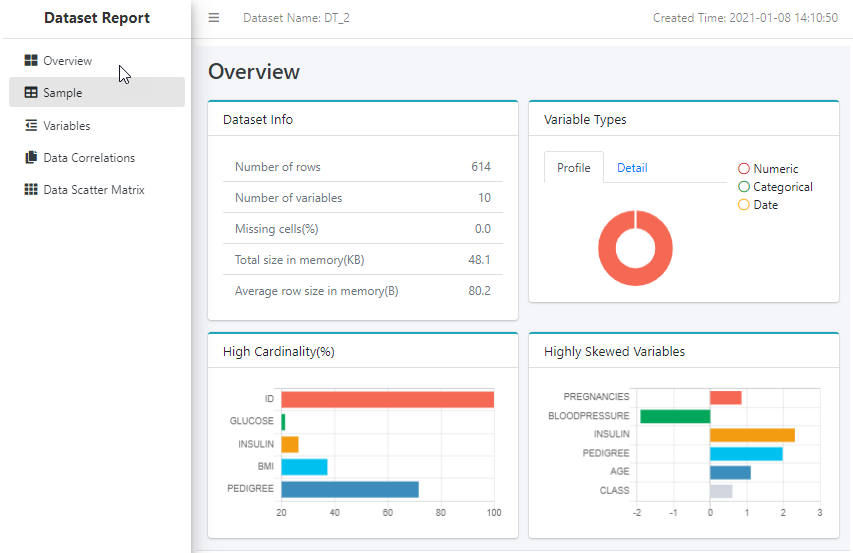Methods

 `build`(data, key[, scatter_matrix_sampling, ...]) Build a report for dataset. `generate_html_report`(filename) Save the dataset report as a html file. Render the dataset report as a notebook iframe. Return the iframe report. Return the html report.
build(data, key, scatter_matrix_sampling: = None, ignore_scatter_matrix: bool = False, ignore_correlation: bool = False)

Build a report for dataset.

Note that the name of data is used as the dataset name in this function. If the name of data (which is a dataframe.DataFrame object) is not set explicitly in the object instantiation, a name like 'DT_XX' will be assigned to the data.

Parameters

DataFrame to use to build the dataset report.

keystr

Name of ID column.

scatter_matrix_sampling`Sampling`, optional

Scatter matrix sampling.

ignore_scatter_matrixbool, optional

Skip calculating scatter matrix.

Defaults to False.

ignore_correlationbool, optional

Skip calculating correlation.

Defaults to False.

generate_html_report(filename)

Save the dataset report as a html file.

Parameters
filenamestr

Html file name.

generate_notebook_iframe_report()

Render the dataset report as a notebook iframe.

get_report_html()

Return the html report.

get_iframe_report_html()

Return the iframe report.

## hana_ml.visualizers.shap¶

This module provides some explainers for Shapley values.

The following classes are available:

class hana_ml.visualizers.shap.ShapleyExplainer(reason_code_data: hana_ml.dataframe.DataFrame, feature_data: hana_ml.dataframe.DataFrame, reason_code_column_name=None, **kwargs)

Bases: `object`

SHAP (SHapley Additive exPlanations) is a game theoretic approach to explain the output of machine learning model.

It connects optimal credit allocation with local explanations using the classic Shapley values from game theory and their related extensions.

To get an overview of which features are most important for a model we can plot the Shapley values of every feature for every sample.

If the output table contains the reason code column, the output table can be parsed by this class in most cases, rather than only valid for the tree model.

Parameters

The Dataframe containing only reason code values.

The Dataframe containing only feature values.

Examples

In the following example, training data is called diabetes_train and test data is diabetes_test.

First, we create an UnifiedClassification instance:

```>>> uc_hgbdt = UnifiedClassification('HybridGradientBoostingTree')
```

Then, create a GridSearchCV instance:

```>>> gscv = GridSearchCV(estimator=uc_hgbdt,
param_grid={'learning_rate': [0.1, 0.4, 0.7, 1],
'n_estimators': [4, 6, 8, 10],
'split_threshold': [0.1, 0.4, 0.7, 1]},
train_control=dict(fold_num=5,
resampling_method='cv',
random_state=1,
ref_metric=['auc']),
scoring='error_rate')
```

Call the fit() function to train the model:

```>>> gscv.fit(data=diabetes_train, key= 'ID',
label='CLASS',
partition_method='stratified',
partition_random_state=1,
stratified_column='CLASS',
build_report=True)
>>> features = diabetes_train.columns
>>> features.remove('CLASS')
>>> features.remove('ID')
```

Use diabetes_test for prediction:

```>>> pred_res = gscv.predict(diabetes_test, key='ID', features=features)
```

Create a ShapleyExplainer class and then invoke summary_plot() :

```>>> shapley_explainer = ShapleyExplainer(reason_code_data=pred_res.select('REASON_CODE'), feature_data=diabetes_test.select(features))
>>> shapley_explainer.summary_plot()
```

Output: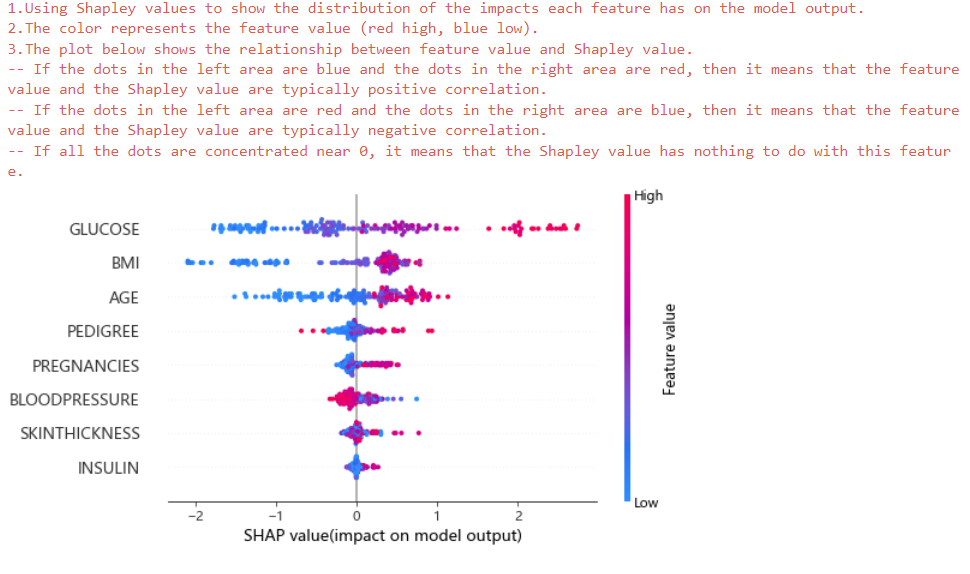Methods

 Renders the force plot as a notebook iframe. Get Shapley values. Global Interpretation using Shapley values.
shap_values()

Get Shapley values.

Returns
numpy.ndarray

Shapley values.

force_plot()

Renders the force plot as a notebook iframe.

summary_plot()

Global Interpretation using Shapley values.

To get an overview of which features are most important for a model we can plot the Shapley values of every feature for every sample.

Returns
Image Component

This object can be rendered by browser.

class hana_ml.visualizers.shap.TimeSeriesExplainer

Bases: `object`

The TimeSeriesExplainer instance can visualize the training and prediction results of time series.

The generated html can be embedded in a notebook, including:

• Compare

• YHAT

• YHAT_LOWER

• YHAT_UPPER

• REAL_Y

• Trend

• Seasonal

• Holiday

• Exogenous variable

Methods

 `explain_additive_model`(amf[, iframe_height]) The static method can visualize the training and prediction results of AdditiveModelForecast. `explain_arima_model`(arima[, iframe_height]) The static method can visualize the training and prediction results of ARIMA.
static explain_arima_model(arima, iframe_height=800)

The static method can visualize the training and prediction results of ARIMA.

The generated html can be embedded in a notebook, including:

• Compare

• PREDICTIVE_Y

• REAL_Y

• Trend

• Seasonal

• Holiday

• Exogenous variable

Parameters
arima :

ARIMA related instances.

iframe_heightint, optional

Specifies iframe height.

Defaults to 800.

The static method can visualize the training and prediction results of AdditiveModelForecast.

The generated html can be embedded in a notebook, including:

• Compare

• YHAT

• YHAT_LOWER

• YHAT_UPPER

• REAL_Y

• Trend

• Seasonal

• Holiday

• Exogenous variable

Parameters

iframe_heightint, optional

Specifies iframe height.

Defaults to 800.

## hana_ml.visualizers.unified_report¶

This module is to build report for PAL/APL models.

The following class is available:

class hana_ml.visualizers.unified_report.UnifiedReport(obj)

Bases: `object`

The report generator for PAL/APL models. Currently, it only supports UnifiedClassification and UnifiedRegression.

Examples

Data used is called diabetes_train.

Case 1: UnifiedReport for UnifiedClassification is shown as follows, please set build_report=True in the fit() function:

```>>> from hana_ml.algorithms.pal.model_selection import GridSearchCV
>>> from hana_ml.algorithms.pal.model_selection import RandomSearchCV
>>> hgc = UnifiedClassification('HybridGradientBoostingTree')
>>> gscv = GridSearchCV(estimator=hgc,
param_grid={'learning_rate': [0.1, 0.4, 0.7, 1],
'n_estimators': [4, 6, 8, 10],
'split_threshold': [0.1, 0.4, 0.7, 1]},
train_control=dict(fold_num=5,
resampling_method='cv',
random_state=1,
ref_metric=['auc']),
scoring='error_rate')
>>> gscv.fit(data=diabetes_train, key= 'ID',
label='CLASS',
partition_method='stratified',
partition_random_state=1,
stratified_column='CLASS',
build_report=True)
```

To look at the dataset report:

```>>> UnifiedReport(diabetes_train).build().display()
```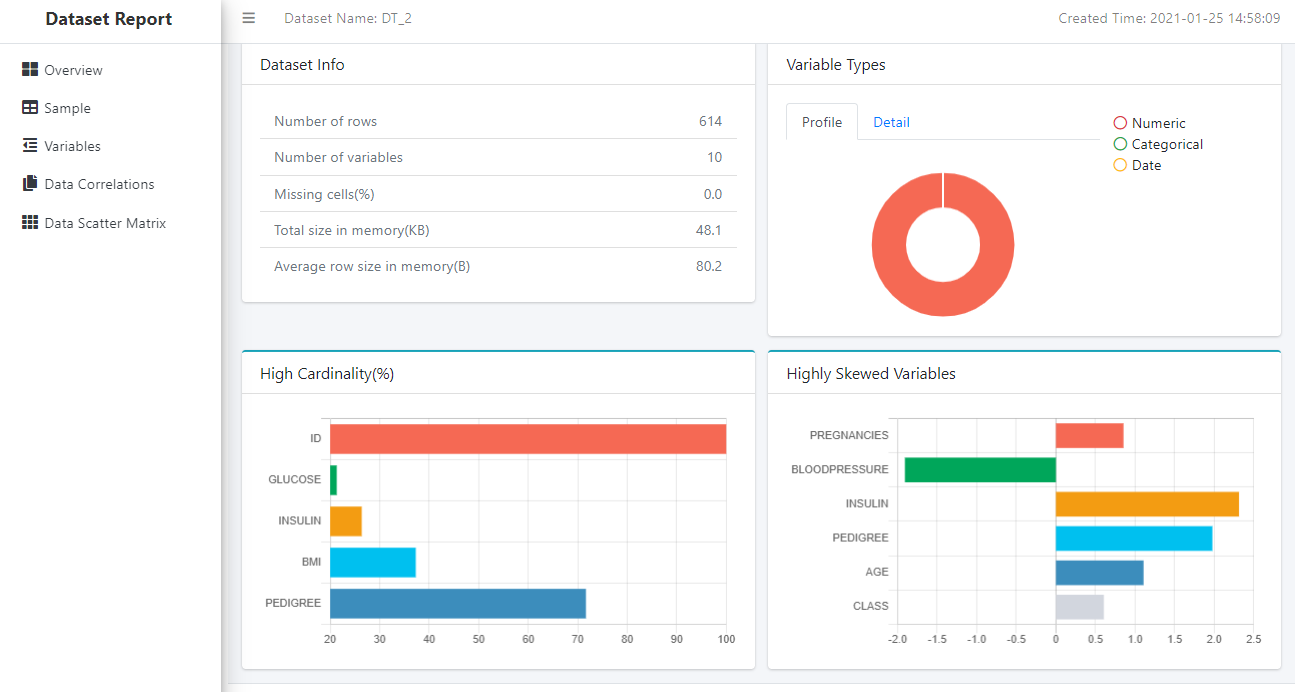To see the model report:

```>>> UnifiedReport(gscv.estimator).display()
```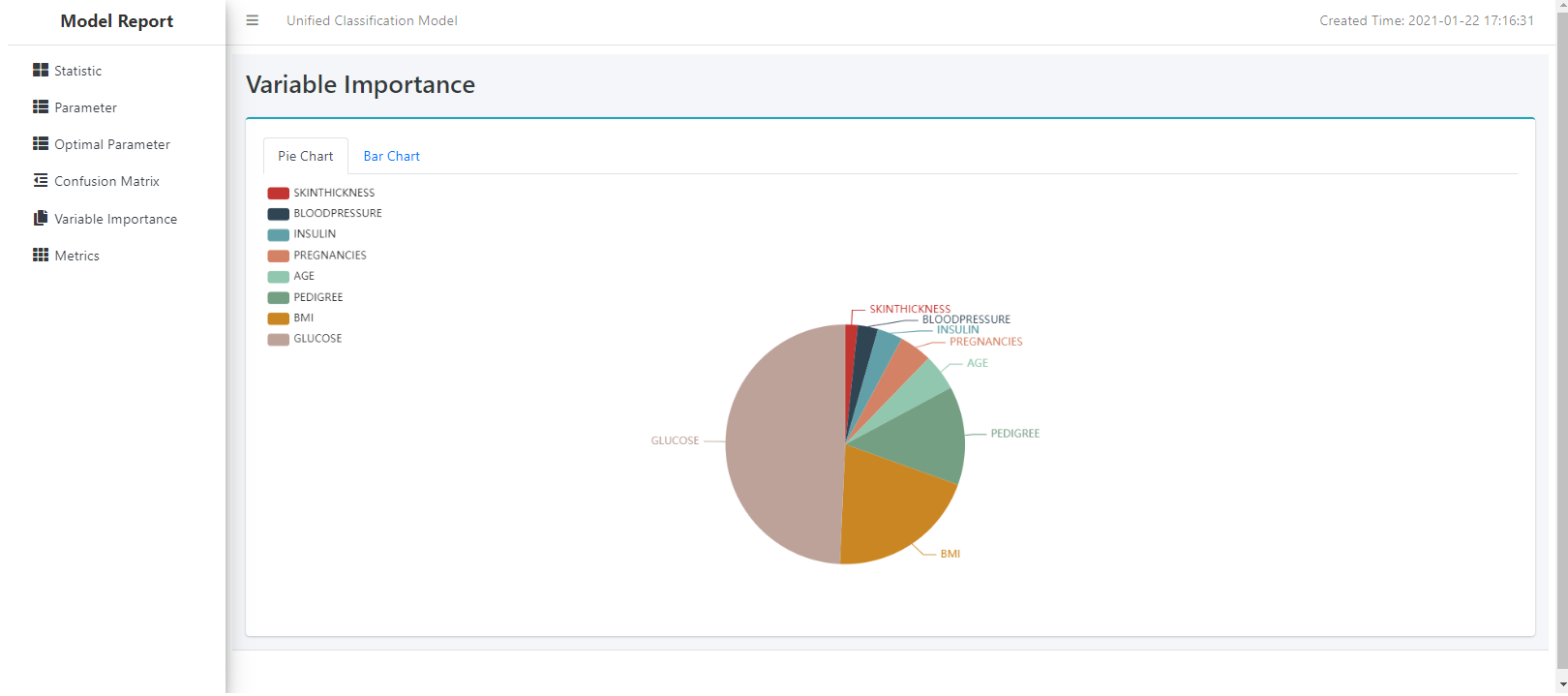We could also see the Optimal Parameter page: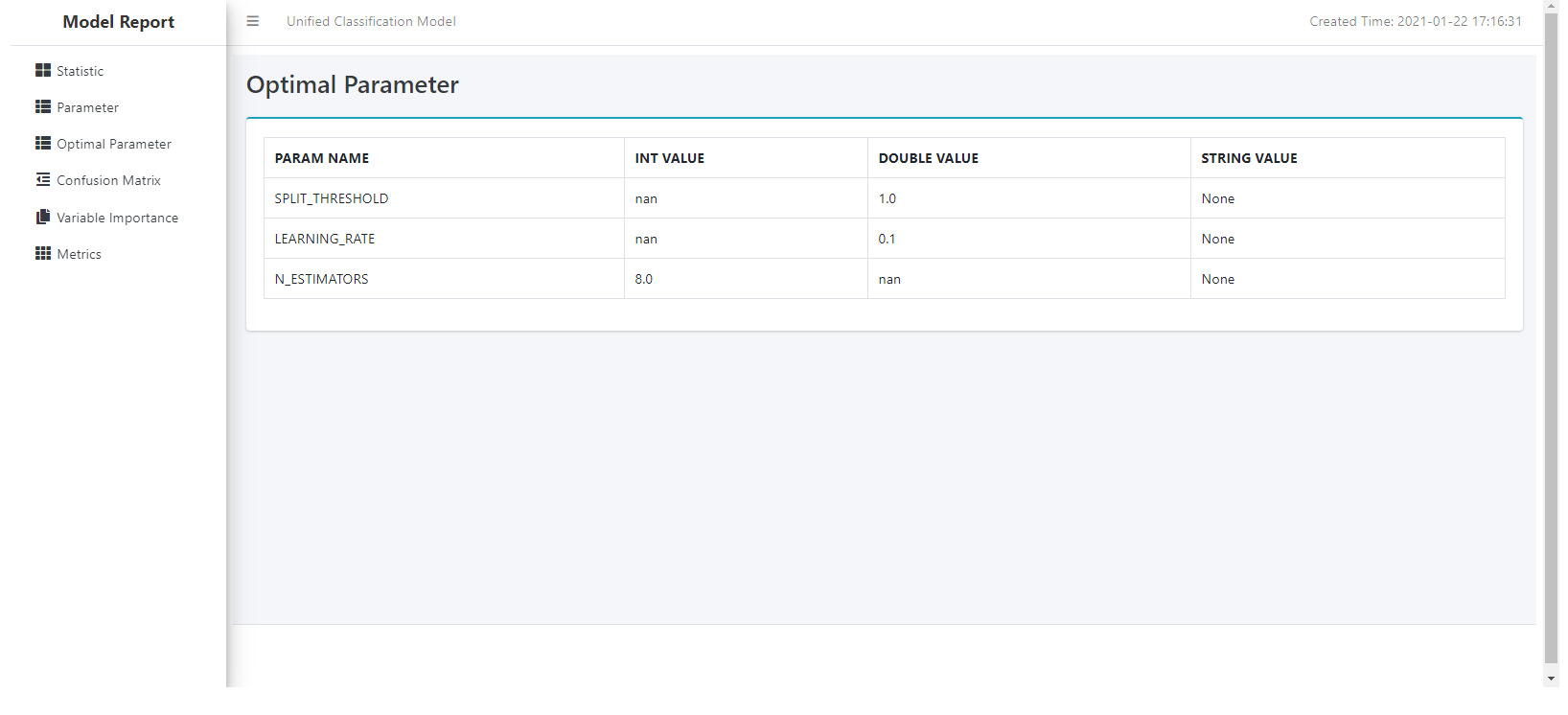Case 2: UnifiedReport for UnifiedRegression is shown as follows, please set build_report=True in the fit() function:

```>>> hgr = UnifiedRegression(func = 'HybridGradientBoostingTree')
>>> gscv = GridSearchCV(estimator=hgr,
param_grid={'learning_rate': [0.1, 0.4, 0.7, 1],
'n_estimators': [4, 6, 8, 10],
'split_threshold': [0.1, 0.4, 0.7, 1]},
train_control=dict(fold_num=5,
resampling_method='cv',
random_state=1),
scoring='rmse')
>>> gscv.fit(data=diabetes_train, key= 'ID',
label='CLASS',
partition_method='random',
partition_random_state=1,
build_report=True)
```

To see the model report:

```>>> UnifiedReport(gscv.estimator).display()
```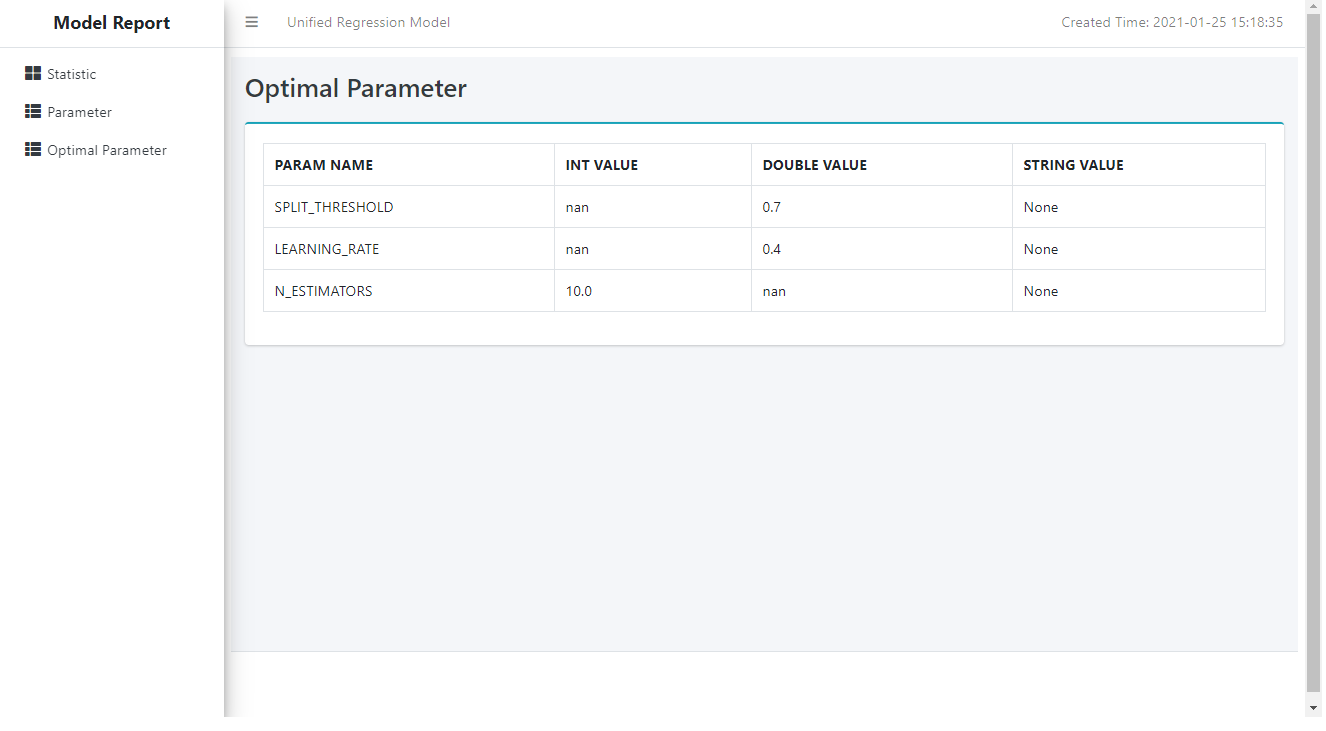Methods

 `build`([key, scatter_matrix_sampling, ...]) Build the report. `display`([save_html, metric_sampling]) Display the report. `set_metric_samplings`([roc_sampling, ...]) Set metric samplings to report builder.
build(key=None, scatter_matrix_sampling: = None, ignore_scatter_matrix: bool = False, ignore_correlation: bool = False)

Build the report.

Parameters
keystr, valid only for DataFrame

Name of ID column.

Defaults to the first column.

scatter_matrix_sampling`Sampling`, valid only for DataFrame

Scatter matrix sampling.

ignore_scatter_matrixbool, optional

Ignore the plotting of scatter matrix if True.

Defaults to False.

ignore_correlationbool, optional

Ignore the correlation computation if True.

Defaults to False.

set_metric_samplings(roc_sampling: = None, other_samplings: Optional[dict] = None)

Set metric samplings to report builder.

Parameters
roc_sampling`Sampling`, optional

ROC sampling.

other_samplingsdict, optional

Key is column name of metric table.

• CUMGAINS

• RANDOM_CUMGAINS

• PERF_CUMGAINS

• LIFT

• RANDOM_LIFT

• PERF_LIFT

• CUMLIFT

• RANDOM_CUMLIFT

• PERF_CUMLIFT

Value is sampling.

Examples

Creating the metric samplings:

```>>> roc_sampling = Sampling(method='every_nth', interval=2)
```
```>>> other_samplings = dict(CUMGAINS=Sampling(method='every_nth', interval=2),
LIFT=Sampling(method='every_nth', interval=2),
CUMLIFT=Sampling(method='every_nth', interval=2))
>>> unified_report.set_metric_samplings(roc_sampling, other_samplings)
```
display(save_html=None, metric_sampling=False)

Display the report.

Parameters
save_htmlstr, optional

If it is not None, the function will generate a html report and stored in the given name.

Defaults to None.

metric_samplingbool, optional (deprecated)

Whether the metric table needs to be sampled. It is only valid for UnifiedClassification and used together with set_metric_samplings. Since version 2.14, the metric_sampling is no need to specify.

Defaults to False.

## hana_ml.visualizers.visualizer_base¶

The following function is available:

hana_ml.visualizers.visualizer_base.forecast_line_plot(pred_data, actual_data=None, confidence=None, ax=None, figsize=None, max_xticklabels=10, marker=None, enable_plotly=False)

Plot the prediction data for time series forecast or regression model.

Parameters

The forecast data to be plotted.

The actual data to be plotted.

Default value is None.

confidencetuple of str, optional

The column names of confidence bound.

Default value is None.

axmatplotlib.Axes, optional

The axes to use to plot the figure. Default value : Current axes

figsizetuple, optional

(weight, height) of the figure. For matplotlib, the unit is inches, and for plotly, the unit is pixels.

Defaults to (15, 12) when using matplotlib, auto when using plotly.

max_xticklabelsint, optional

The maximum number of xtick labels. Defaults to 10.

marker: character, optional

Type of maker on the plot.

Default to None indicates no marker.

enable_plotlybool, optional

Use plotly instead of matplotlib.

Defaults to False.

Examples

Create an 'AdditiveModelForecast' instance and invoke the fit and predict functions:

```>>> amf = AdditiveModelForecast(growth='linear')
>>> amf.fit(data=train_df)
>>> pred_data = amf.predict(data=test_df)
```

Visualize the forecast values:

```>>> ax = forecast_line_plot(pred_data=pred_data.set_index("INDEX"),
actual_data=df.set_index("INDEX"),
confidence=("YHAT_LOWER", "YHAT_UPPER"),
max_xticklabels=10)
```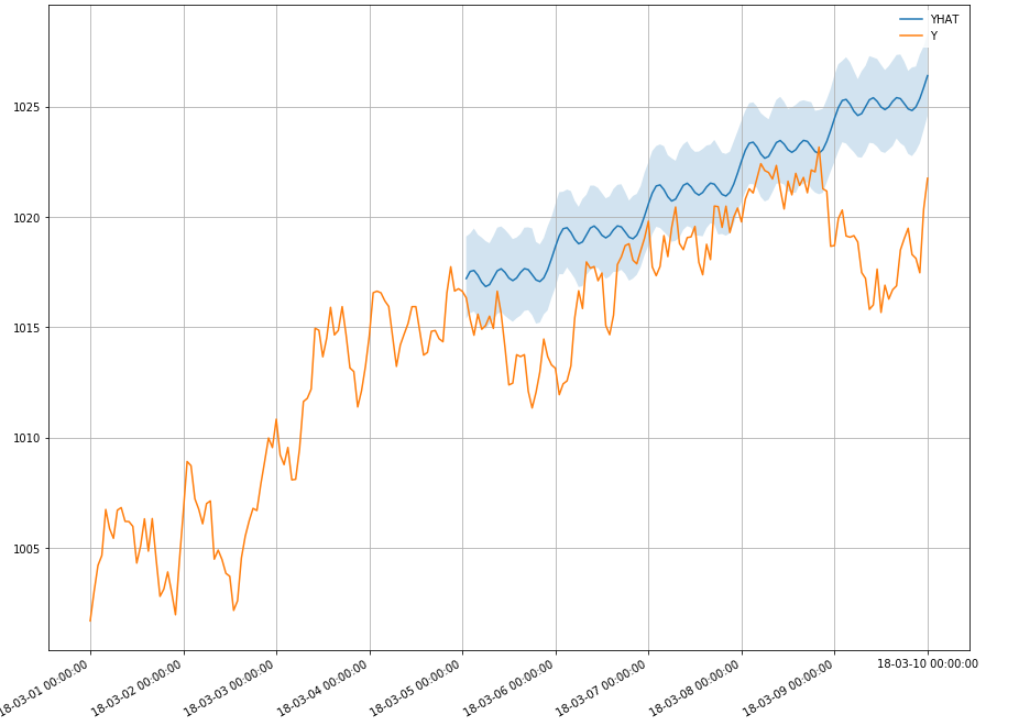## hana_ml.visualizers.digraph¶

This module represents the whole digraph framework. The whole digraph framework consists of Python API and page assets(HTML, CSS, JS, Font, Icon, etc.). The application scenarios of the current digraph framework are AutoML Pipeline and Model Debriefing.

The following classes are available:
class hana_ml.visualizers.digraph.Node(node_id: int, node_name: str, node_icon_id: int, node_content: str, node_in_ports: list, node_out_ports: list)

Bases: `object`

The Node class of digraph framework is an entity class.

Parameters
node_idint [Automatic generation]

Unique identification of node.

node_namestr

The node name.

node_icon_idint [Automatic generation]

Unique identification of node icon.

node_contentstr

The node content.

node_in_portslist

List of input port names.

node_out_portslist

List of output port names.

class hana_ml.visualizers.digraph.InPort(node: hana_ml.visualizers.digraph.Node, port_id: str, port_name: str, port_sequence: int)

Bases: `object`

The InPort class of digraph framework is an entity class.

A port is a fixed connection point on a node.

Parameters
nodeNode

Which node is the input port fixed on.

port_idstr [Automatic generation]

Unique identification of input port.

port_namestr

The input port name.

port_sequenceint [Automatic generation]

The position of input port among all input ports.

class hana_ml.visualizers.digraph.OutPort(node: hana_ml.visualizers.digraph.Node, port_id: str, port_name: str, port_sequence: int)

Bases: `object`

The OutPort class of digraph framework is an entity class.

A port is a fixed connection point on a node.

Parameters
nodeNode

Which node is the output port fixed on.

port_idstr [Automatic generation]

Unique identification of output port.

port_namestr

The output port name.

port_sequenceint [Automatic generation]

The position of output port among all output ports.

class hana_ml.visualizers.digraph.Edge(source_port: hana_ml.visualizers.digraph.OutPort, target_port: hana_ml.visualizers.digraph.InPort)

Bases: `object`

The Edge class of digraph framework is an entity class.

The output port of a node is connected with the input port of another node to make an edge.

Parameters
source_portOutPort

Start connection point of edge.

target_portInPort

End connection point of edge.

class hana_ml.visualizers.digraph.DigraphConfig

Bases: `object`

Configuration class of digraph.

Methods

 `set_digraph_layout`([digraph_layout]) Set the layout of a digraph. `set_node_sep`([node_sep]) Set distance between nodes. `set_rank_sep`([rank_sep]) Set distance between layers. `set_text_layout`([make_text_center]) Set node"s text layout.
set_text_layout(make_text_center: bool = False)

Set node"s text layout.

Parameters
make_text_centerbool, optional

Should the node"s text be centered.

Defaults to False.

set_digraph_layout(digraph_layout: str = 'horizontal')

Set the layout of a digraph.

Parameters
digraph_layoutstr, optional

The layout of a digraph can only be horizontal or vertical.

Defaults to horizontal layout.

set_node_sep(node_sep: int = 80)

Set distance between nodes.

Under horizontal layout, this parameter represents horizontal distance between nodes.

Under vertical layout, this parameter represents vertical distance between nodes.

Parameters
node_sepint, optional

The distance between nodes.

The value range of parameter is 20 to 200.

Defaults to 80.

set_rank_sep(rank_sep: int = 80)

Set distance between layers.

Under horizontal layout, this parameter represents vertical distance between nodes.

Under vertical layout, this parameter represents horizontal distance between nodes.

Parameters
rank_sepint, optional

The distance between layers.

The value range of parameter is 20 to 200.

Defaults to 80.

class hana_ml.visualizers.digraph.Digraph(digraph_name: str, embedded_mode: bool = False)

Bases: `hana_ml.visualizers.digraph.BaseDigraph`

Using the Digraph class of digraph framework can dynamically add nodes and edges, and finally generate an HTML page. The rendered HTML page can display the node information and the relationship between nodes, and provide a series of auxiliary tools to help you view the digraph. A series of auxiliary tools are provided as follows:

• Provide basic functions such as pan and zoom.

• Locate the specified node by keyword search.

• Look at the layout outline of the whole digraph through the minimap.

• Through the drop-down menu to switch different digraph.

• The whole page can be displayed in full screen.

• Adjust the distance between nodes and distance between layers dynamically.

• Provide the function of node expansion and collapse.

Parameters
digraph_namestr

The digraph name.

Examples

1. Importing classes of digraph framework

```>>> from hana_ml.visualizers.digraph import Digraph, Node, Edge
```
1. Creating a Digraph instance:

```>>> digraph: Digraph = Digraph("Test1")
```
1. Adding two nodes to digraph instance, where the node1 has only one output port and the node2 has only one input port:

```>>> node1: Node = digraph.add_model_node("name1", "content1", in_ports=[], out_ports=["1"])
>>> node2: Node = digraph.add_python_node("name2", "content2", in_ports=["1"], out_ports=[])
```
1. Adding an edge to digraph instance, where the output port of node1 points to the input port of node2:

```>>> edge1_2: Edge = digraph.add_edge(node1.out_ports, node2.in_ports)
```
1. Create a DigraphConfig instance:

```>>> digraph_config = DigraphConfig()
>>> digraph_config.set_digraph_layout("vertical")
```
1. Generating notebook iframe:

```>>> digraph.build(digraph_config)
>>> digraph.generate_notebook_iframe(iframe_height=500)
```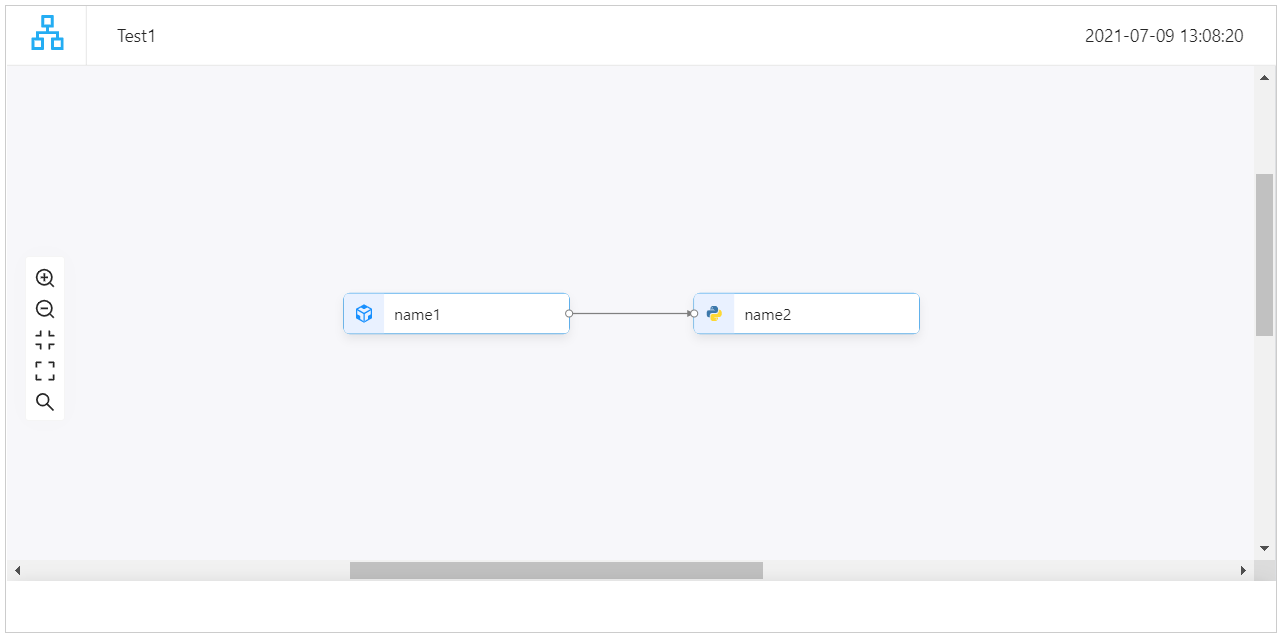1. Generating a local HTML file:

```>>> digraph.generate_html("Test1")
```

Methods

 `add_edge`(source_port, target_port) Add edge to digraph instance. `add_model_node`(name, content, in_ports, ...) Add node with model icon to digraph instance. `add_python_node`(name, content, in_ports, ...) Add node with python icon to digraph instance. `build`([digraph_config]) Build HTML string based on current data. `generate_html`(filename) Save the digraph as a html file. `generate_notebook_iframe`([iframe_height]) Render the digraph as a notebook iframe. Return the nodes and edges data of digraph.
to_json() list

Return the nodes and edges data of digraph.

Returns
list

The nodes and edges data of digraph.

build(digraph_config: = None)

Build HTML string based on current data.

Parameters
digraph_configDigraphConfig, optional

Configuration instance of digraph.

generate_html(filename: str)

Save the digraph as a html file.

Parameters
filenamestr

HTML file name.

generate_notebook_iframe(iframe_height: int = 800)

Render the digraph as a notebook iframe.

add_edge(source_port: hana_ml.visualizers.digraph.OutPort, target_port: hana_ml.visualizers.digraph.InPort)

Add edge to digraph instance.

Parameters
source_portOutPort

Start connection point of edge.

target_portInPort

End connection point of edge.

Returns
Edge

add_model_node(name: str, content: str, in_ports: list, out_ports: list)

Add node with model icon to digraph instance.

Parameters
namestr

The model node name.

contentstr

The model node content.

in_portslist

List of input port names.

out_portslist

List of output port names.

Returns
Node

The added node with model icon.

add_python_node(name: str, content: str, in_ports: List, out_ports: List)

Add node with python icon to digraph instance.

Parameters
namestr

The python node name.

contentstr

The python node content.

in_portslist

List of input port names.

out_portslist

List of output port names.

Returns
Node

The added node with python icon.

class hana_ml.visualizers.digraph.MultiDigraph(multi_digraph_name: str, embedded_mode: bool = False)

Bases: `object`

Using the MultiDigraph class of digraph framework can dynamically add multiple child digraphs, and finally generate an HTML page. The rendered HTML page can display the node information and the relationship between nodes, and provide a series of auxiliary tools to help you view the digraph. A series of auxiliary tools are provided as follows:

• Provide basic functions such as pan and zoom.

• Locate the specified node by keyword search.

• Look at the layout outline of the whole digraph through the minimap.

• Through the drop-down menu to switch different digraph.

• The whole page can be displayed in fullscreen.

• Adjust the distance between nodes and distance between layers dynamically.

• Provide the function of node expansion and collapse.

Parameters
multi_digraph_namestr

The digraph name.

Examples

1. Importing classes of digraph framework

```>>> from hana_ml.visualizers.digraph import MultiDigraph, Node, Edge
```
1. Creating a MultiDigraph instance:

```>>> multi_digraph: MultiDigraph = MultiDigraph("Test2")
```
1. Creating first digraph:

```>>> digraph1 = multi_digraph.add_child_digraph("digraph1")
```
1. Adding two nodes to digraph1, where the node1_1 has only one output port and the node2_1 has only one input port:

```>>> node1_1: Node = digraph1.add_model_node("name1", "content1", in_ports=[], out_ports=["1"])
>>> node2_1: Node = digraph1.add_python_node("name2", "content2", in_ports=["1"], out_ports=[])
```
1. Adding an edge to digraph1, where the output port of node1_1 points to the input port of node2_1:

```>>> digraph1.add_edge(node1_1.out_ports, node2_1.in_ports)
```
1. Creating second digraph:

```>>> digraph2 = multi_digraph.add_child_digraph("digraph2")
```
1. Adding two nodes to digraph2, where the node1_2 has only one output port and the node2_2 has only one input port:

```>>> node1_2: Node = digraph2.add_model_node("name1", "model text", in_ports=[], out_ports=["1"])
>>> node2_2: Node = digraph2.add_python_node("name2", "function info", in_ports=["1"], out_ports=[])
```
1. Adding an edge to digraph2, where the output port of node1_2 points to the input port of node2_2:

```>>> digraph2.add_edge(node1_2.out_ports, node2_2.in_ports)
```
1. Generating notebook iframe:

```>>> multi_digraph.build()
>>> multi_digraph.generate_notebook_iframe(iframe_height=500)
```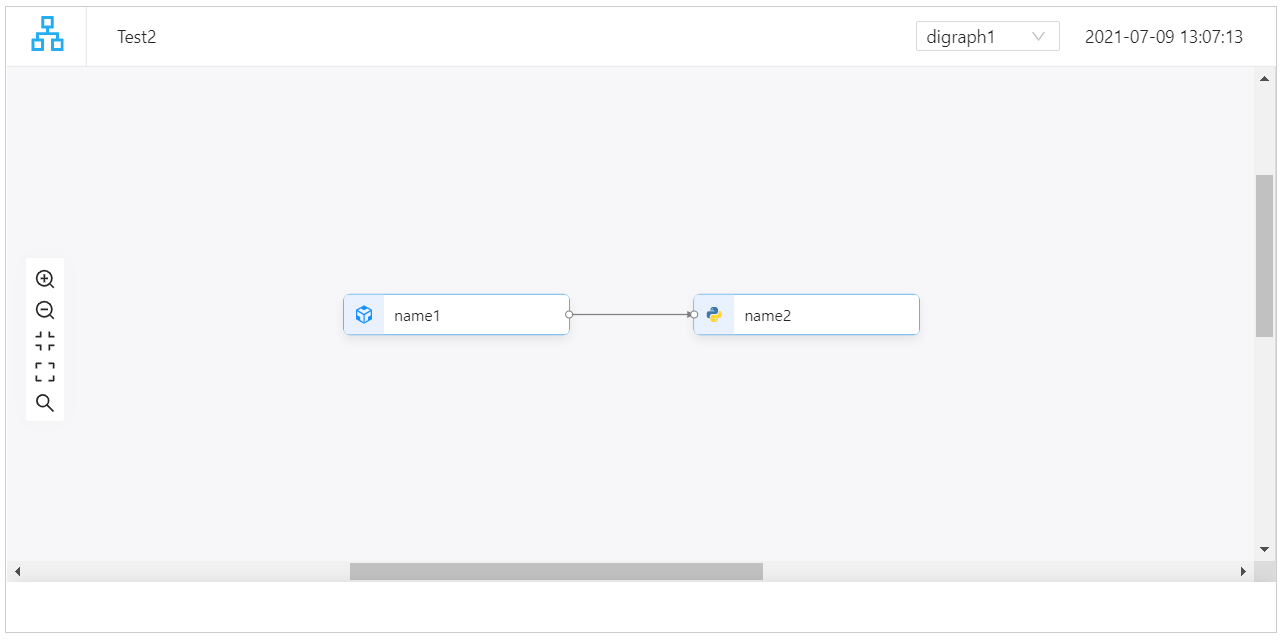1. Generating a local HTML file:

```>>> multi_digraph.generate_html("Test2")
```

Methods

 `ChildDigraph`(child_digraph_id, ...) Multiple child digraphs are logically a whole. `add_child_digraph`(child_digraph_name) Add child digraph to multi_digraph instance. `build`([digraph_config]) Build HTML string based on current data. `generate_html`(filename) Save the digraph as a html file. `generate_notebook_iframe`([iframe_height]) Render the digraph as a notebook iframe. Return the nodes and edges data of whole digraph.
class ChildDigraph(child_digraph_id: int, child_digraph_name: str, embedded_mode: bool = False)

Bases: `hana_ml.visualizers.digraph.BaseDigraph`

Multiple child digraphs are logically a whole.

Methods

 `add_edge`(source_port, target_port) Add edge to digraph instance. `add_model_node`(name, content, in_ports, ...) Add node with model icon to digraph instance. `add_python_node`(name, content, in_ports, ...) Add node with python icon to digraph instance. Return the nodes and edges data of child digraph.
to_json() list

Return the nodes and edges data of child digraph.

Returns
list

The nodes and edges data of whole digraph.

add_edge(source_port: hana_ml.visualizers.digraph.OutPort, target_port: hana_ml.visualizers.digraph.InPort)

Add edge to digraph instance.

Parameters
source_portOutPort

Start connection point of edge.

target_portInPort

End connection point of edge.

Returns
Edge

add_model_node(name: str, content: str, in_ports: list, out_ports: list)

Add node with model icon to digraph instance.

Parameters
namestr

The model node name.

contentstr

The model node content.

in_portslist

List of input port names.

out_portslist

List of output port names.

Returns
Node

The added node with model icon.

add_python_node(name: str, content: str, in_ports: List, out_ports: List)

Add node with python icon to digraph instance.

Parameters
namestr

The python node name.

contentstr

The python node content.

in_portslist

List of input port names.

out_portslist

List of output port names.

Returns
Node

The added node with python icon.

Add child digraph to multi_digraph instance.

Parameters
child_digraph_namestr

The child digraph name.

Returns
ChildDigraph

The added child digraph.

to_json() list

Return the nodes and edges data of whole digraph.

Returns
list

The nodes and edges data of whole digraph.

build(digraph_config: = None)

Build HTML string based on current data.

Parameters
digraph_configDigraphConfig, optional

Configuration instance of digraph.

generate_html(filename: str)

Save the digraph as a html file.

Parameters
filenamestr

Html file name.

generate_notebook_iframe(iframe_height: int = 800)

Render the digraph as a notebook iframe.

## hana_ml.visualizers.word_cloud¶

WordCloud Visualization.

The following classes and functions are available:

class hana_ml.visualizers.word_cloud.WordCloud(font_path=None, width=400, height=200, margin=2, ranks_only=None, prefer_horizontal=0.9, mask=None, scale=1, color_func=None, max_words=200, min_font_size=4, stopwords=None, random_state=None, background_color='black', max_font_size=None, font_step=1, mode='RGB', relative_scaling='auto', regexp=None, collocations=True, colormap=None, normalize_plurals=True, contour_width=0, contour_color='black', repeat=False, include_numbers=False, min_word_length=0, collocation_threshold=30)

Bases: `wordcloud.wordcloud.WordCloud`

Extended from wordcloud.WordCloud.

Methods

 `build`(data[, content_column]) Generate wordcloud. `fit_words`(frequencies) Create a word_cloud from words and frequencies. `generate`(text) Generate wordcloud from text. `generate_from_frequencies`(frequencies[, ...]) Create a word_cloud from words and frequencies. Generate wordcloud from text. `process_text`(text) Splits a long text into words, eliminates the stopwords. `recolor`([random_state, color_func, colormap]) Recolor existing layout. Convert to numpy array. `to_file`(filename) Export to image file. `to_svg`([embed_font, optimize_embedded_font, ...]) Export to SVG.
build(data, content_column=None)

Generate wordcloud.

Parameters

The input SAP HANA DataFrame.

content_columnstr, optional

Specified the column to do wordcloud. Defaults to the first column.

Examples

```>>> wordcloud = WordCloud(background_color="white", max_words=2000,
max_font_size=100, random_state=42, width=1000,
height=860, margin=2).build(data, content_column="CONTENT")
>>> import matplotlib.pyplot as plt
>>> plt.imshow(wordcloud, interpolation='bilinear')
>>> plt.axis("off")
```
fit_words(frequencies)

Create a word_cloud from words and frequencies.

Alias to generate_from_frequencies.

Parameters
frequenciesdict from string to float

A contains words and associated frequency.

Returns
self
generate(text)

Generate wordcloud from text.

The input "text" is expected to be a natural text. If you pass a sorted list of words, words will appear in your output twice. To remove this duplication, set `collocations=False`.

Alias to generate_from_text.

Calls process_text and generate_from_frequencies.

Returns
self
generate_from_frequencies(frequencies, max_font_size=None)

Create a word_cloud from words and frequencies.

Parameters
frequenciesdict from string to float

A contains words and associated frequency.

max_font_sizeint

Use this font-size instead of self.max_font_size

Returns
self
generate_from_text(text)

Generate wordcloud from text.

The input "text" is expected to be a natural text. If you pass a sorted list of words, words will appear in your output twice. To remove this duplication, set `collocations=False`.

Calls process_text and generate_from_frequencies.

..versionchanged:: 1.2.2

Argument of generate_from_frequencies() is not return of process_text() any more.

Returns
self
process_text(text)

Splits a long text into words, eliminates the stopwords.

Parameters
textstring

The text to be processed.

Returns
wordsdict (string, int)

Word tokens with associated frequency.

..versionchanged:: 1.2.2

Changed return type from list of tuples to dict.

recolor(random_state=None, color_func=None, colormap=None)

Recolor existing layout.

Applying a new coloring is much faster than generating the whole wordcloud.

Parameters
random_stateRandomState, int, or None, default=None

If not None, a fixed random state is used. If an int is given, this is used as seed for a random.Random state.

color_funcfunction or None, default=None

Function to generate new color from word count, font size, position and orientation. If None, self.color_func is used.

colormapstring or matplotlib colormap, default=None

Use this colormap to generate new colors. Ignored if color_func is specified. If None, self.color_func (or self.color_map) is used.

Returns
self
to_array()

Convert to numpy array.

Returns
imagend-array size (width, height, 3)

Word cloud image as numpy matrix.

to_file(filename)

Export to image file.

Parameters
filenamestring

Location to write to.

Returns
self
to_svg(embed_font=False, optimize_embedded_font=True, embed_image=False)

Export to SVG.

Font is assumed to be available to the SVG reader. Otherwise, text coordinates may produce artifacts when rendered with replacement font. It is also possible to include a subset of the original font in WOFF format using `embed_font` (requires fontTools).

Note that some renderers do not handle glyphs the same way, and may differ from `to_image` result. In particular, Complex Text Layout may not be supported. In this typesetting, the shape or positioning of a grapheme depends on its relation to other graphemes.

Pillow, since version 4.2.0, supports CTL using `libraqm`. However, due to dependencies, this feature is not always enabled. Hence, the same rendering differences may appear in `to_image`. As this rasterized output is used to compute the layout, this also affects the layout generation. Use `PIL.features.check` to test availability of `raqm`.

Consistant rendering is therefore expected if both Pillow and the SVG renderer have the same support of CTL.

Contour drawing is not supported.

Parameters
embed_fontbool, default=False

Whether to include font inside resulting SVG file.

optimize_embedded_fontbool, default=True

Whether to be aggressive when embedding a font, to reduce size. In particular, hinting tables are dropped, which may introduce slight changes to character shapes (w.r.t. to_image baseline).

embed_imagebool, default=False

Whether to include rasterized image inside resulting SVG file. Useful for debugging.

Returns
contentstring

Word cloud image as SVG string

## hana_ml.visualizers.automl_progress¶

This module contains related classes for monitoring the pipeline progress status.

The following class is available:

class hana_ml.visualizers.automl_progress.PipelineProgressStatusMonitor(connection_context: hana_ml.dataframe.ConnectionContext, automatic_obj, interval=0.01)

Bases: `object`

The instance of this class can monitor the progress of AutoML execution.

Parameters
connection_context`ConnectionContext`

The connection to the SAP HANA system. Please use a new connection to SAP HANA when you create a new PipelineProgressStatusMonitor object.

For example:

progress_status_monitor = PipelineProgressStatusMonitor(connection_context=dataframe.ConnectionContext(url, port, user, pwd), automatic_obj=auto_c)

automatic_obj

An instance object of the AutomaticClassification type or AutomaticRegression type that contains the progress_indicator_id attribute.

intervalfloat, optional

Specifies the time interval of updating the UI of pipeline progress.

Defaults to 0.01s.

Examples

Create an AutomaticClassification instance:

```>>> progress_id = "automl_{}".format(uuid.uuid1())
>>> auto_c = AutomaticClassification(generations=2,
population_size=5,
offspring_size=5,
progress_indicator_id=progress_id)
```

Invoke a PipelineProgressStatusMonitor:

```>>> progress_status_monitor = PipelineProgressStatusMonitor(connection_context=dataframe.ConnectionContext(url, port, user, pwd),
automatic_obj=auto_c)
>>> progress_status_monitor.start()
>>> auto_c.fit(data=df_train)
```

Output: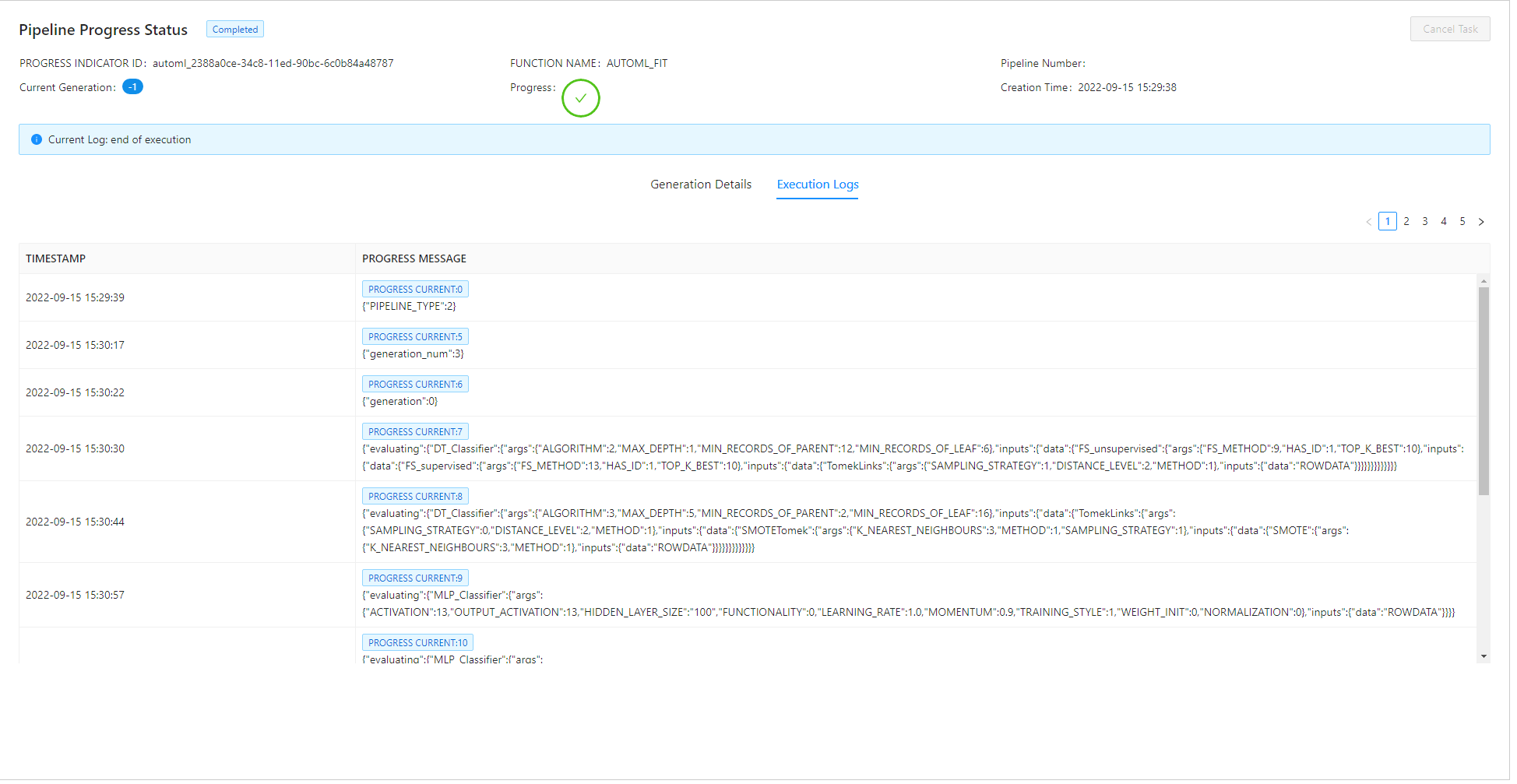Methods

 Call the method before executing the fit method of AutomaticClassification or AutomaticRegression.
start()

Call the method before executing the fit method of AutomaticClassification or AutomaticRegression.

## hana_ml.visualizers.automl_report¶

This module contains related class for generating the best pipeline report.

The following class is available:

class hana_ml.visualizers.automl_report.BestPipelineReport(automatic_obj)

Bases: `object`

The instance of this class can generate the best pipeline report.

Parameters
automatic_obj

An instance object of the AutomaticClassification type or AutomaticRegression type.

Examples

Create an AutomaticClassification instance:

```>>> progress_id = "automl_{}".format(uuid.uuid1())
>>> auto_c = AutomaticClassification(generations=2,
population_size=5,
offspring_size=5,
progress_indicator_id=progress_id)
```

Training:

```>>> auto_c.fit(data=df_train)
```

Plot the best pipeline:

```>>> BestPipelineReport(auto_c).generate_notebook_iframe()
```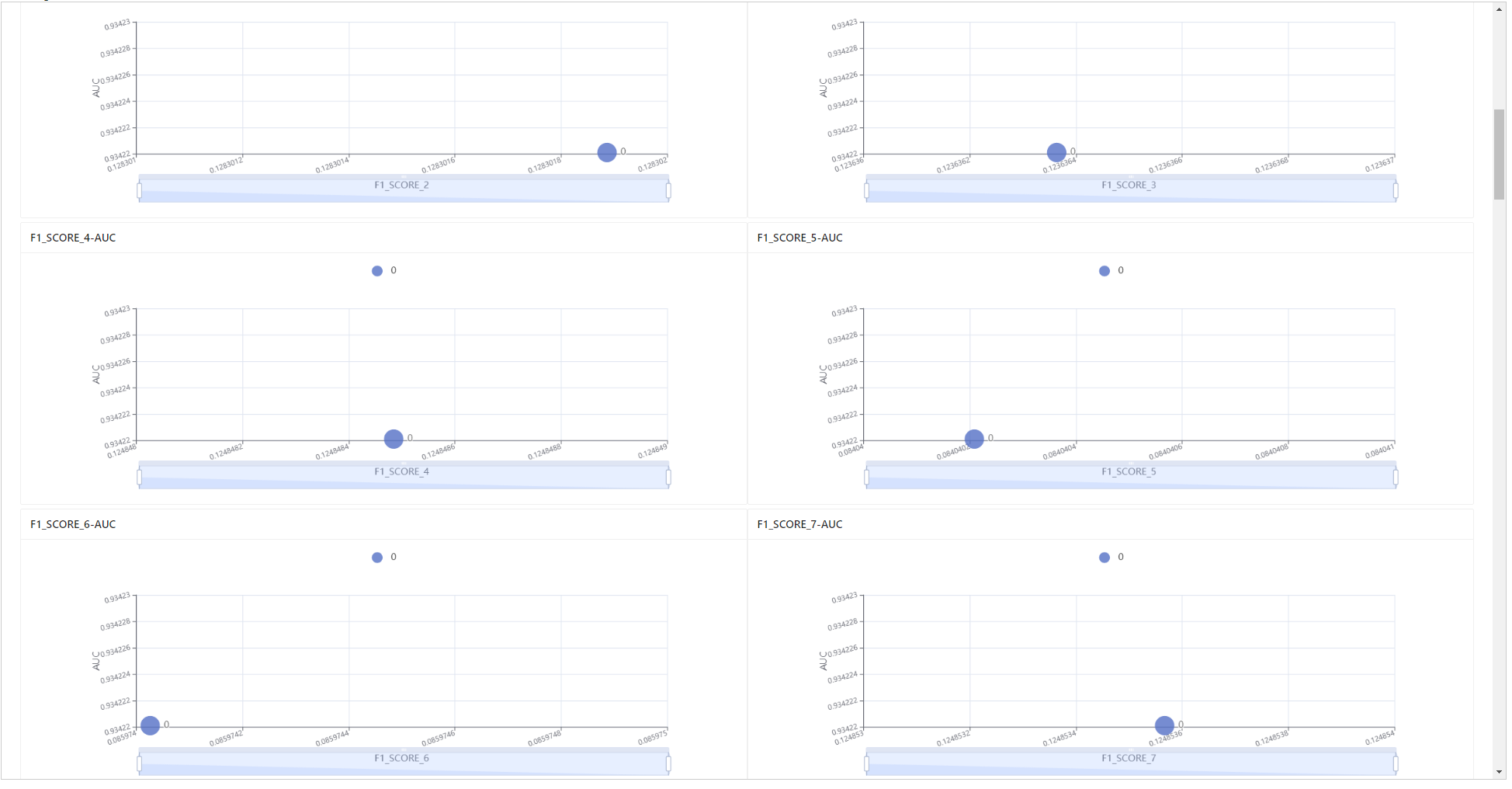Methods

 `generate_html`(filename) Saves the best pipeline report as a html file. `generate_notebook_iframe`([iframe_height]) Renders the best pipeline report as a notebook iframe.
generate_notebook_iframe(iframe_height: int = 1000)

Renders the best pipeline report as a notebook iframe.

Parameters
iframe_heightint, optional

Frame height.

Defaults to 1000.

generate_html(filename: str)

Saves the best pipeline report as a html file.

Parameters
filenamestr

Html file name.

## hana_ml.visualizers.time_series_report¶

This module represents the whole time series report. A report can contain many pages, and each page can contain many items. You can use the class 'DatasetAnalysis' to generate all the items and combine them into different pages at will.

The following classes are available:
class hana_ml.visualizers.time_series_report.TimeSeriesReport(title: str)

Bases: `hana_ml.visualizers.report_builder.ReportBuilder`

This class is the builder of time series report.

Parameters
titlestr

The name of time series report.

Examples

1. Importing classes

```>>> from hana_ml.visualizers.time_series_report import TimeSeriesReport, DatasetAnalysis
>>> from hana_ml.visualizers.report_builder import Page
```
1. Creating a report instance:

```>>> report = TimeSeriesReport('Time Series Data Report')
```
1. Create a data analysis instance and a page array:

```>>> dataset_analysis = DatasetAnalysis(data=df_acf, endog="Y", key="ID")
>>> pages = []
```
1. Construct the contents of each page of the report:

```>>> page0 = Page('Stationarity')
>>> pages.append(page0)
```
```>>> page1 = Page('Partial Autocorrelation')
>>> pages.append(page1)
```
```>>> page2 = Page('Rolling Mean and Standard Deviation')
>>> pages.append(page2)
```
```>>> page3 = Page('Real and Seasonal')
>>> pages.append(page3)
```
```>>> page4 = Page('Box')
>>> pages.append(page4)
```
```>>> page5 = Page('Quarter')
>>> pages.append(page5)
```
```>>> page6 = Page('Outlier')
>>> pages.append(page6)
```
1. Add all pages to report instance:

```>>> report.addPages(pages)
```
1. Generating notebook iframe:

```>>> report.build()
>>> report.generate_notebook_iframe()
```
1. Generating a local HTML file:

```>>> report.generate_html("TimeSeriesReport")
```

Methods

 `addPage`(page) Add a page instance to report instance. `addPages`(pages) Add many page instances to report instance. `build`([debug]) Build HTML string based on current config. `generate_html`(filename) Save the report as a html file. `generate_notebook_iframe`([iframe_height]) Render the report as a notebook iframe. Return the all config data of report.

Add a page instance to report instance.

Parameters
pagePage

Every report consists of many pages.

Add many page instances to report instance.

Parameters
pagesList[Page]

Every report consists of many pages.

build(debug=False)

Build HTML string based on current config.

Parameters
debugbool

Whether the log should be printed to the console.

Defaults to False.

generate_html(filename)

Save the report as a html file.

Parameters
filenamestr

HTML file name.

generate_notebook_iframe(iframe_height=600)

Render the report as a notebook iframe.

Parameters
iframe_heightint

iframe height.

Defaults to 600.

to_json()

Return the all config data of report. This method is automatically called by the internal framework.

class hana_ml.visualizers.time_series_report.DatasetAnalysis(data, endog, key=None)

Bases: `object`

This class will generate all items of dataset analysis result.

Parameters

Input data.

endogstr

Name of the dependent variable.

keystr, optional

Name of the ID column.

Defaults to the index column of `data` (i.e. data.index) if it is set.

Methods

 `moving_average_item`(rolling_window) It will plot rolling mean by given rolling window size. `outlier_item`([window_size, ...]) Perform PAL time series outlier detection and plot time series with the highlighted outliers. `pacf_item`([thread_ratio, method, max_lag, ...]) It will plot PACF for two time series data. It performs quarter plot to view the seasonality. It will plot a chart based on the original data. `rolling_stddev_item`(rolling_window) It will plot rolling standard deviation by given rolling window size. `seasonal_decompose_items`([alpha, ...]) It will to decompose a time series into three components: trend, seasonality and random noise, then to plot. It will plot time series data by year. `stationarity_item`([method, mode, lag, ...]) Stationarity means that a time series has a constant mean and constant variance over time. `timeseries_box_item`([cycle]) It will plot year-wise/month-wise box plot.
pacf_item(thread_ratio=None, method=None, max_lag=None, calculate_confint=True, alpha=None, bartlett=None)

It will plot PACF for two time series data.

Parameters
colstr

Name of the time series data column.

The ratio of available threads.

• 0: single thread

• 0~1: percentage

• Others: heuristically determined

Valid only when `method` is set as 'brute_force'.

Defaults to -1.

method{'auto', 'brute_force', 'fft'}, optional

Indicates the method to be used to calculate the correlation function.

Defaults to 'auto'.

max_lagint, optional

Maximum lag for the correlation function.

calculate_confintbool, optional

Controls whether to calculate confidence intervals or not.

If it is True, two additional columns of confidence intervals are shown in the result.

Defaults to True.

alphafloat, optional

Confidence bound for the given level are returned. For instance if alpha=0.05, 95 % confidence bound is returned.

Valid only when only `calculate_confint` is True.

Defaults to 0.05.

bartlettbool, optional
• False: using standard error to calculate the confidence bound.

• True: using Bartlett's formula to calculate confidence bound.

Valid only when only `calculate_confint` is True.

Defaults to True.

Returns
itemChartItem

The item for the plot.

moving_average_item(rolling_window)

It will plot rolling mean by given rolling window size.

Parameters
rolling_windowint, optional

Window size for rolling function. If negative, it will use the points before CURRENT ROW.

Returns
itemChartItem

The item for the plot.

rolling_stddev_item(rolling_window)

It will plot rolling standard deviation by given rolling window size.

Parameters
rolling_windowint, optional

Window size for rolling function. If negative, it will use the points before CURRENT ROW.

Returns
itemChartItem

The item for the plot.

seasonal_item()

It will plot time series data by year.

Returns
itemChartItem

The item for the plot.

timeseries_box_item(cycle=None)

It will plot year-wise/month-wise box plot.

Parameters
cycle{"YEAR", "QUARTER", "MONTH", "WEEK"}, optional

It defines the x-axis for the box plot.

Returns
itemChartItem

The item for the plot.

seasonal_decompose_items(alpha=None, thread_ratio=None, decompose_type=None, extrapolation=None, smooth_width=None)

It will to decompose a time series into three components: trend, seasonality and random noise, then to plot.

Parameters
alphafloat, optional

The criterion for the autocorrelation coefficient. The value range is (0, 1). A larger value indicates stricter requirement for seasonality.

Defaults to 0.2.

Controls the proportion of available threads to use. The ratio of available threads.

• 0: single thread.

• 0~1: percentage.

• Others: heuristically determined.

Defaults to -1.

decompose_type{'additive', 'multiplicative', 'auto'}, optional

Specifies decompose type. - 'additive': Additive decomposition model - 'multiplicative': Multiplicative decomposition model - 'auto': Decomposition model automatically determined from input data

Defaults to 'auto'.

extrapolationbool, optional

Specifies whether to extrapolate the endpoints. Set to True when there is an end-point issue.

Defaults to False.

smooth_widthint, optional

Specifies the width of the moving average applied to non-seasonal data. 0 indicates linear fitting to extract trends. Can not be larger than half of the data length.

Defaults to 0.

Returns
itemChartItem

The item for the plot.

quarter_item()

It performs quarter plot to view the seasonality.

Returns
itemChartItem

The item for the plot.

outlier_item(window_size=None, detect_seasonality=None, alpha=None, periods=None, outlier_method=None, threshold=None)

Perform PAL time series outlier detection and plot time series with the highlighted outliers.

Parameters
window_sizeint, optional

Odd number, the window size for median filter, not less than 3.

Defaults to 3.

outlier_methodstr, optional

The method for calculate the outlier score from residual. - 'z1' : Z1 score. - 'z2' : Z2 score. - 'iqr' : IQR score. - 'mad' : MAD score.

Defaults to 'z1'.

thresholdfloat, optional

The threshold for outlier score. If the absolute value of outlier score is beyond the threshold, we consider the corresponding data point as an outlier.

Defaults to 3.

detect_seasonalitybool, optional

When calculating the residual,

• False: Does not consider the seasonal decomposition.

• True: Considers the seasonal decomposition.

Defaults to False.

alphafloat, optional

The criterion for the autocorrelation coefficient. The value range is (0, 1). A larger value indicates a stricter requirement for seasonality.

Only valid when `detect_seasonality` is True.

Defaults to 0.2.

periodsint, optional

When this parameter is not specified, the algorithm will search the seasonal period. When this parameter is specified between 2 and half of the series length, autocorrelation value is calculated for this number of periods and the result is compared to `alpha` parameter. If correlation value is equal to or higher than `alpha`, decomposition is executed with the value of `periods`. Otherwise, the residual is calculated without decomposition. For other value of parameter `periods`, the residual is also calculated without decomposition.

No Default value.

The ratio of available threads.

• 0: single thread.

• 0~1: percentage.

• Others: heuristically determined.

Only valid when `detect_seasonality` is True.

Defaults to -1.

Returns
itemChartItem

The item for the plot.

stationarity_item(method=None, mode=None, lag=None, probability=None)

Stationarity means that a time series has a constant mean and constant variance over time. For many time series models, the input data has to be stationary for reasonable analysis.

Parameters
methodstr, optional

Statistic test that used to determine stationarity. The options are "kpss" and "adf".

Defaults "kpss".

modestr, optional

Type of stationarity to determine. The options are "level", "trend" and "no". Note that option "no" is not applicable to "kpss".

Defaults to "level".

lagint, optional

The lag order to calculate the test statistic.

Default value is "kpss": int(12*(data_length / 100)^0.25" ) and "adf": int(4*(data_length / 100)^(2/9)).

probabilityfloat, optional

The confidence level for confirming stationarity.

Defaults to 0.9.

Returns
itemTableItem

The item for the statistical data.

real_item()

It will plot a chart based on the original data.

Returns
itemChartItem

The item for the plot.